• 【转】关于邦迪（J.A. Bondy）的图论教材
千次阅读
2009-08-27 20:32:00
 http://bbs.math.org.cn/viewthread.php?tid=360   我想很多学习图论的人都知道J.A. Bondy和U.S.R. Murty著的《Graph Theory with Application》(Elsevier,1976)是图论教材中的经典，时至今日，仍不失为初学者较好的入门书。还记得兰州交通大学的张忠辅教授说过，国内第一届图论学会就是把大家集中起来学习邦迪的《Graph Theory with Application》，由此可见这本书对国内图论届的影响是如此之大。吴望名等人将其译成中文版本《图论及其应用》（北京：科学出版社，1984），1988年张克民等人编写了该书的参考答案《图论及其应用习题解答》（清华大学出版社，1988）。     在2008年J.A. Bondy和U.S.R. Murty出了新书《Graph Theory》(GTM 244, Springer, 2008), 大家可不妨将其看成是《Graph Theory with Application》的第二版，这本书在内容上做了重新调整，毕竟在第一版出版后的近30年里涌现出了很多新的结果，所以《Graph Theory》在内容上加进了一些新的结果，这本书我只是读了其中的几章，觉得写的非常棒，建议大家能够读读，这里也值得一提的是将第一版最后提出的50个问题进行了更新，并补充了一些新的问题。总之，我个人认为，《Graph Theory》的确是一部很优秀的图论教材。下面给出这两部教材及其答案的链接（在此对资源的提供者表示感谢，如果下列链接失效，请自行baidu或者google）：1. 《Graph Theory with Application》英文版下载：http://old.math.org.cn/forums/index.php?showtopic=57282http://www.ecp6.jussieu.fr/pageperso/bondy/books/gtwa/gtwa.html2. 《Graph Theory with Application》中文版下载：http://old.math.org.cn/forums/index.php?showtopic=548713. 《Graph Theory with Application》答案下载：http://old.math.org.cn/forums/index.php?showtopic=548784.  《Graph Theory》下载：http://ifile.it/5kdc19/1846289696.pdf.zipgraph google
更多相关内容
• 图论经典教材 PDF版就是有些印刷质量问题 毕竟是老教材了
• 非常好的一本书，我就是用这个学习的图论。经典教材，希望能对大家有帮助
• 图论及其应用习题答案(J.A.邦迪)，经典教材中译文版本，包含答案
• 本书为图论及其应用（邦迪着）中文版，如果想阅读英文原版，可以根据文件第一页上的邮箱发送邮件索取
• 图论入门教材首选，经典教材，通俗易懂,大学上图论时老师给的教材
• 经典图论教材，附上习题解答，图论入门教材首选，绝对经典
• 图论方面经典教材，中文版的哦，看起来不那么费劲了。
• 学习图论的好帮手，相当齐全的图理论，分章节介绍，详细又经典
• 图论及其应用邦迪著的中文版.pdf 图论及其应用邦迪著的中文版.pdf 还不错
• [图论及其应用]J.A.邦迪，该领域最经典的教材之一，中文翻译版本！
• 图论及其应用（邦迪, Bondy, 默蒂, Murty） 比较有名的图论书，英文版
• 图论及其应用[J.A.Bondy][扫描版].pdf 清晰扫描版，值得拥有
• 这是一本介绍图论的书籍，非常适合图论初学者使用。如果在解压时需要密码，可用解压工具打开密码说明，里面有密码。
• 图论经典教材, 适合计算机系与数学系使用. 图被广泛应用于描述数据结构, 几乎可以用来表现所有类型的结构或系统
• 图论及其应用 / (美)J.A.邦迪(J.A.Bondy),U.S.R. 默蒂(U.S.R.Murty)著; 吴望名等译
• 4、邦迪定理（闭包定理）【充分必要条件】:图G是H图当且仅当它的闭包是H图 5、邦迪-沙瓦达定理（度序列判定法）【充分条件】:设简单图G的度序列是(d1,d2,…,dn), 这里，d1≦d2≦…≦dn,并且n≧3.若对任意的mm,或有...

# 第四章 欧拉图与哈密尔顿图

## (一)、欧拉图及其性质

(1)、问题背景—欧拉与哥尼斯堡七桥问题

问题：对于图G，它在什么条件下满足从某点出发，经过每条边一次且仅一次，可以回到出发点？

注：一笔画----中国古老的民间游戏（存在欧拉迹）

要求：对于一个图G, 笔不离纸, 一笔画成.

拓展：三笔画：在原图上添加三笔，可使其变为欧拉图。

定义1 对于连通图G，如果G中存在经过每条边的闭迹，则称G为欧拉图，简称G为E图。欧拉闭迹又称为欧拉环游，或欧拉回路。

定理1 下列陈述对于非平凡连通图G是等价的：

​ (1) G是欧拉图；

​ (2) G的顶点度数为偶数；

​ (3) G的边集合能划分为圈。

推论1 连通图G是欧拉图当且仅当G的顶点度数为偶。

推论2 连通非欧拉图G存在欧拉迹当且仅当G中只有两个顶点度数为奇数。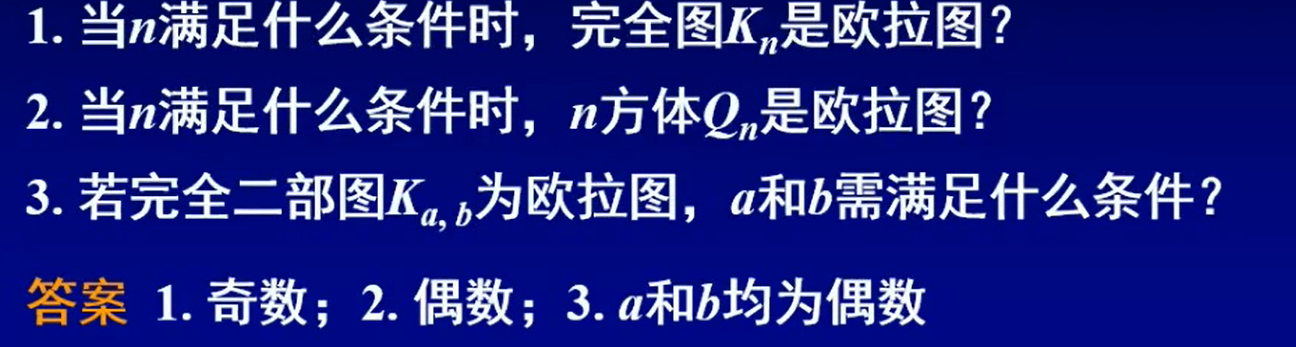证明：若G和H是欧拉图，则 G × H G\times H 是欧拉图。

若G是非平凡的欧拉图，则G的每个块也是欧拉图。## (二)、Fleury算法(欧拉图中求出一条具体欧拉环游的方法)

方法是尽可能避割边行走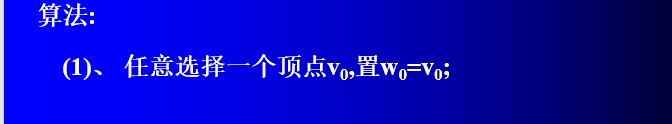## (三)、中国邮路问题（最优欧拉环游，管梅谷）

定理2 若W是包含图G的每条边至少一次的闭途径，则W具有最小权值当且仅当下列两个条件被满足：

(1) G的每条边在W中最多重复一次；

(2) 对于G的每个圈上的边来说，在W中重复的边的总权值不超过该圈非重复边总权值。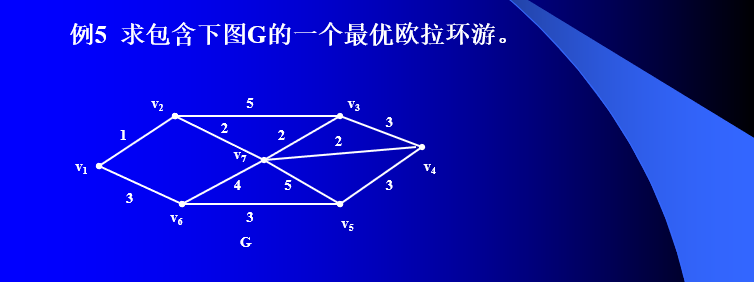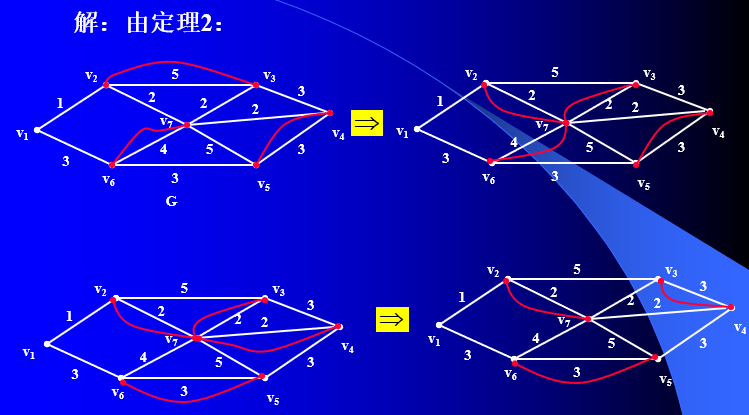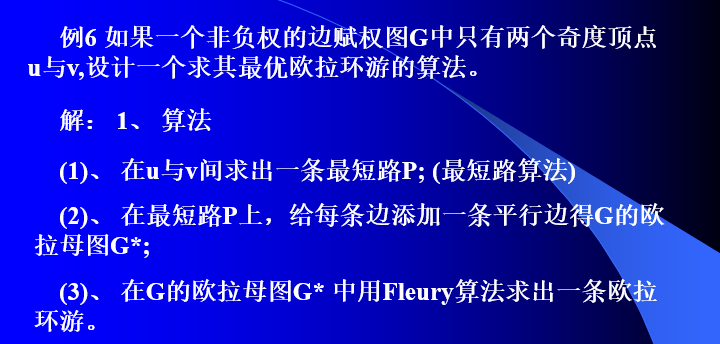## (四)、哈密尔顿图的概念

定义1 ： 如果经过图G的每个顶点恰好一次后能够回到出发点，称这样的图为哈密尔顿图，简称H图。所经过的闭途径是G的一个生成圈，称为G的哈密尔顿圈。

定义2: 如果存在经过G的每个顶点恰好一次的路，称该路为G的哈密尔顿路，简称H路。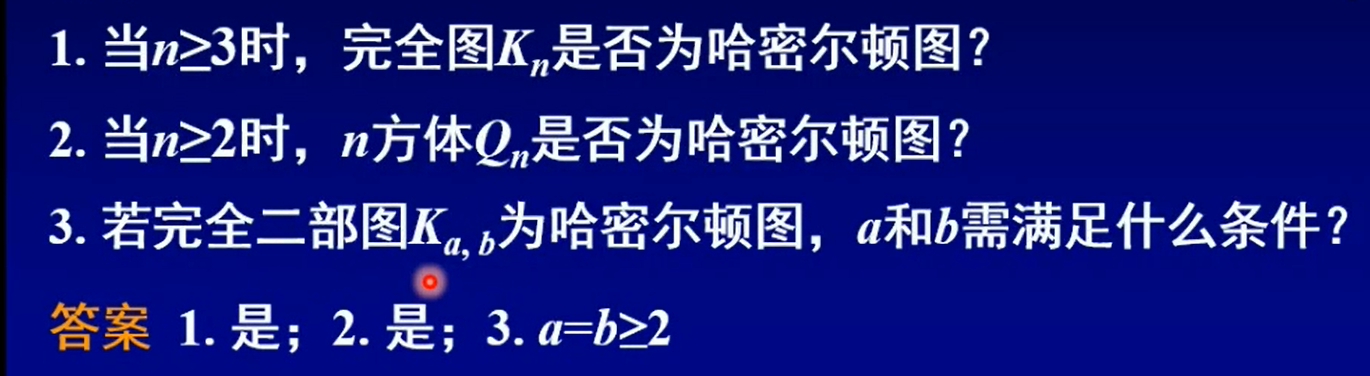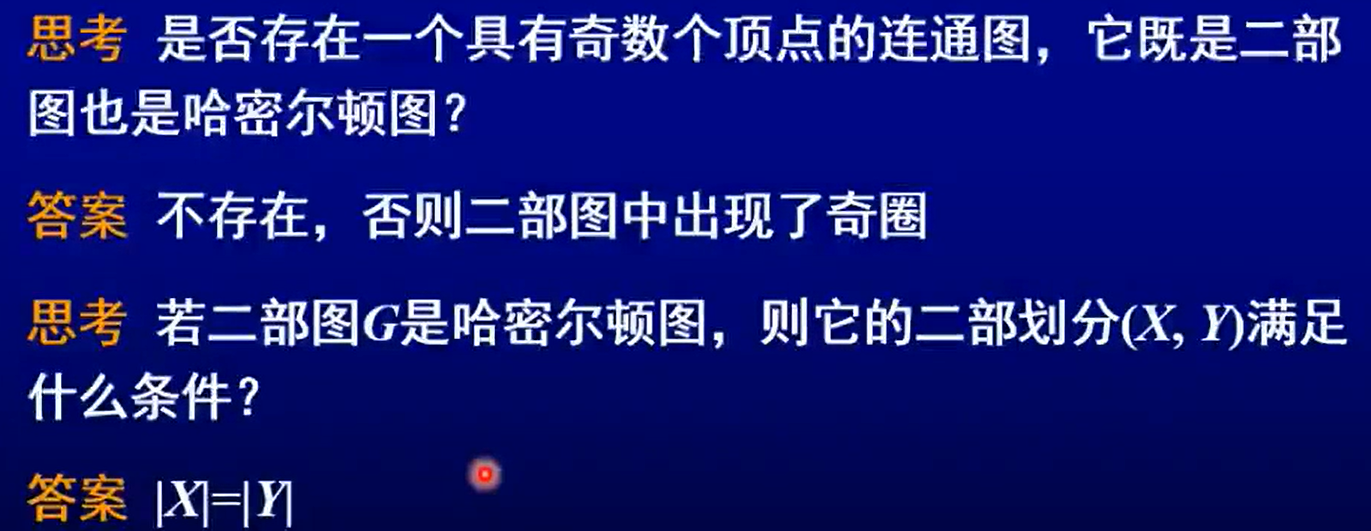## (五)、哈密尔顿图性质与判定

### 1、性质定理【必要条件】；

定理1 (必要条件) 若G为H图，则对V(G)的任一非空顶点子集S，有：
w ( G − S ) ≤ ∣ S ∣ w(G-S)\leq | S|

注：不等式为G是H图的必要条件，即不等式不满足时，可断定对应图是非H、图。满足定理1不等式的图不一定是H图。

著名的彼德森图是非H图

若连通图G不是2-连通的，则G不是哈密尔顿图

### 2、狄拉克定理【充分条件】；

定理2 (充分条件) 对于n≧3的单图G，如果G中有： δ ( G ) ≥ n 2 \delta(G) \geq \frac{n}{2} ,则G是H图.

### 3、奥尔定理【充分条件】；

定理3 (充分条件) 对于n≧3的单图G，如果G中的任意两个不相邻顶点u与v，有：
d ( u ) + d ( v ) ≥ n d(u) + d(v) \geq n
那么，G是H图。

### 4、邦迪定理（闭包定理）【充分必要条件】；

定义3 在n阶单图中，若对d (u) + d (v) ≧n 的任意一对顶点u与v，均有u adj v , 则称G是闭图

引理1 若G1和G2是同一个点集V的两个闭图，则G = G1∩G2是闭图。

注：G1与G2都是闭图，它们的并不一定是闭图。

定义4 如果 G ‾ \overline{G} 是包含G的极小闭图，则其是图G的闭包

图的闭包的构造方法：将图中度数之和至少是图的顶点个数的非邻接顶点对递归地连接起来，直到不再有这样的顶点对存在时为止。

引理2 图G的闭包是唯一的

引理3 对于单图G，如果G中有两个不相邻顶点u与v，满足：
d ( u ) + d ( v ) ≥ n d(u)+d(v) \geq n
那么G是H图当且仅当G + uv是H图 。

定理4(帮迪——闭包定理) 图G是H图当且仅当它的闭包是H图。【引理3证】

说明：1、对于一个具体的图，我们可以作出其闭包，若能够断定闭包是H图，则原图为H图。否则，定理失效！【太麻烦】

​ 2、如果对满足一定条件的图G，能够从逻辑上说明其闭包是H图，则可以断定G是H图【掌握一点，看下面的例子】。

推论1：设G是n≧3的单图，若G的闭包是完全图，则G是H图。

### 5、邦迪-沙瓦达定理（度序列判定法）【充分条件】。

定理5(Chvátal——度序列判定法) 设简单图G的度序列是(d1,d2,…,dn), 这里，d1≦d2≦…≦dn,并且n≧3.若对任意的m<n/2,或有 dm>m,或有dn-m ≧ n-m,则G是H图。【可以证明，满足条件的图的闭包是完全图】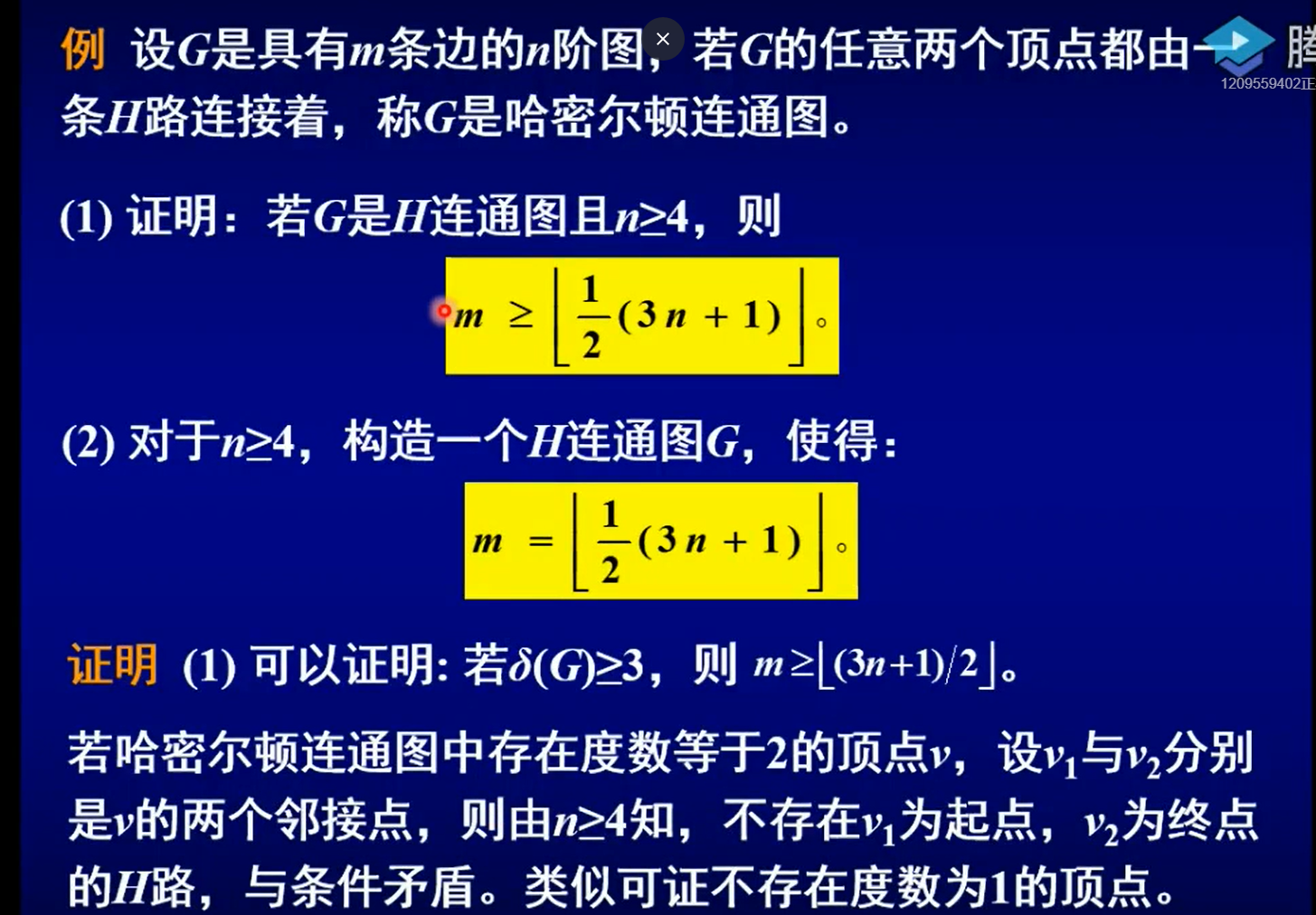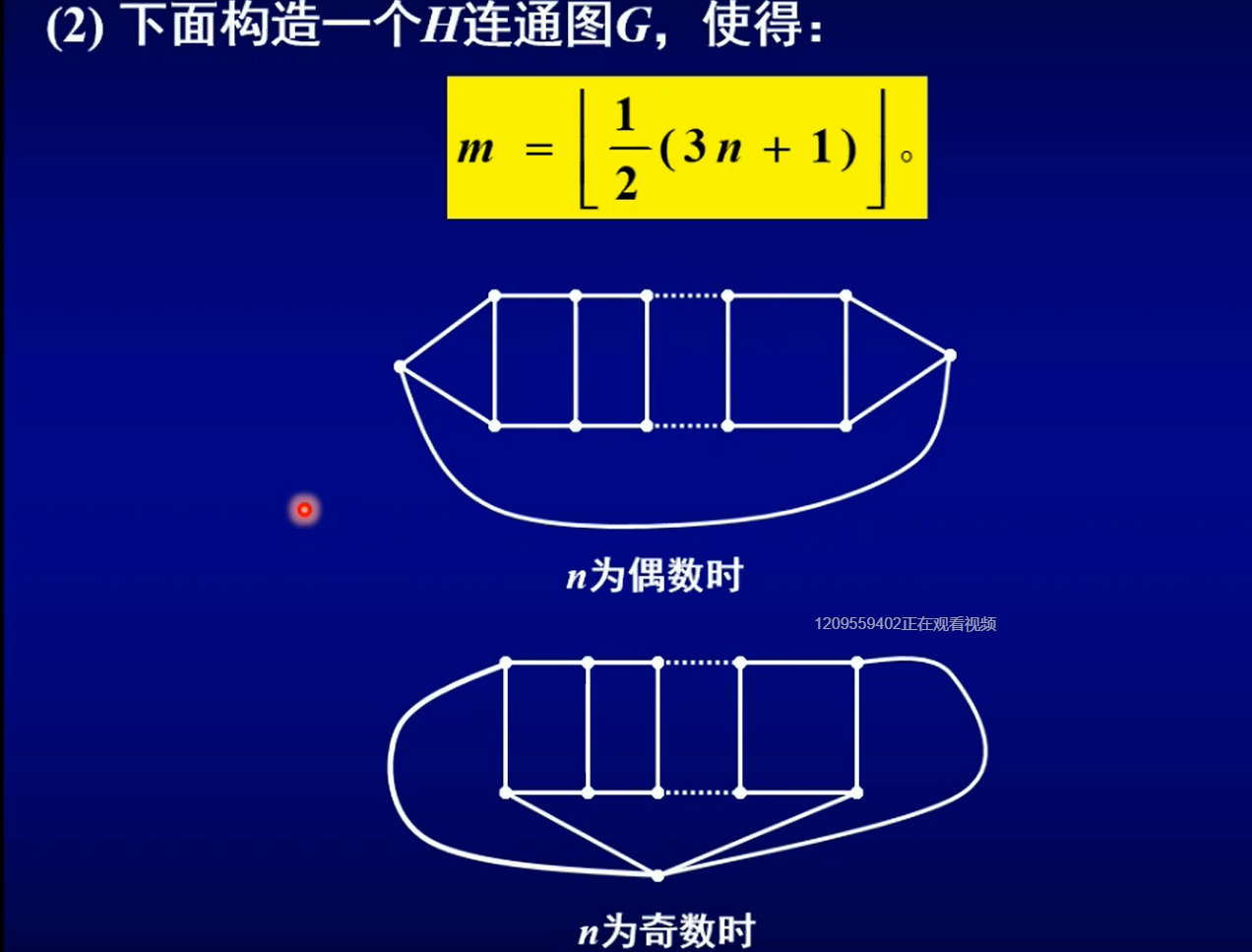### 6、 范定理

​ 若连通图中每对距离为2的点中有一点的度数至少是图的点数的一半，则该图是哈密尔顿图。

### 7、边数判定法

设G是n阶简单图。若n≥3且KaTeX parse error: Undefined control sequence: \matrix at position 14: |E(G)>\left( \̲m̲a̲t̲r̲i̲x̲{{n-1}\\2}\righ…则G是H图；并且具有n个顶点条KaTeX parse error: Undefined control sequence: \matrix at position 8: \left( \̲m̲a̲t̲r̲i̲x̲{{n-1}\\ 2} \ri…边的非H图只有 C 1 , n C_{1,n} 以及 C 2 , 5 C_{2,5}

## (六)、非哈密尔顿图与TSP问题

### 一、非哈密尔顿图特征

定义1 图G称为度极大非H图，如果它的度不弱于其它非H图。

定义2 对于 1 ≤ m ≤ n 2 1\leq m \leq \frac{n}{2} , C m , n C_{m,n} 图定义为：
C m , n = K m ∨ ( K m ‾ + K n − 2 m ) C_{m,n}=K_m \vee(\overline{K_m}+K_{n-2m})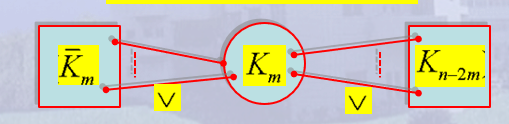引理1 对于 1 ≤ m ≤ n 2 1\leq m \leq \frac{n}{2} , C m , n C_{m,n} 图是非H图。

定理1 (Chvátal,1972) 若G是n≧3的非H单图，则G度弱于某个 C m , n C_{m,n} 图。(性质)

注： (1) 定理1刻画了非H单图的特征：从度序列角度看， C m , n C_{m,n} 图族中某个图是某个n阶非H单图的极图。

(2) C m , n C_{m,n} 图族中的图是度极大非哈密尔顿简单图。

(3) 定理1的逆不能成立！但有结论：n(≥3)阶单图若度优于C m, n 图族中所有图，则G是H图。

推论边数判定法！） 设G是n阶单图。若n≧3且，则G是H图。并且，具有n个顶点条边的非H图只有 C 1 , n C_{1,n} 以及 C 2 , 5 C_{2,5} .

### 二、TSP问题(旅行售货员问题)

在赋权图中求最小H圈是NP—难问题。理论上也已经证明：不存在多项式时间近似算法，使相对误差小于或等于某个固定的常数ε,即便是ε=1000也是如此。

#### **(**一)、边交换技术【赋权完全图中】

注：为了得到进一步的优解，可以从几个不同的初始圈开始，通过边交换技术得到几个近似最优解，然后从中选取一个近似解。

#### (二)、赋权完全图中最优H圈下界估计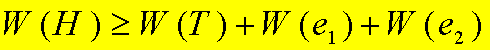## (七)、超哈密尔顿图与超可迹图问题

### (一)、超H图与超可迹图【训练逻辑演绎】

定义1 若图G是非H图，但对于G中任意点v,都有G-v是H图，则称G是超H图。

定理1 彼得森图是超H图。

定义2 若G中没有H路，但是对G中任意点v，G-v存在H路，则称G是超可迹的。

1、加莱猜想：不存在超可迹的图。 错误

2、泰特猜想：任何3连通3正则可平面图是H图。 错误

3、纳什—威廉斯猜想：每个4连通4正则图是H图。 错误

4、托特猜想：每个3连通3正则偶图是H图。 错误

5、普鲁默猜想：每个2连通图的平方是H图。 正确

定义：图G的平方 G 2 G^2 是这样的图: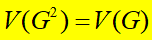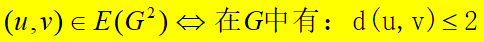#### 1.1 H图中H圈的计数问题。

定理：每个3正则H图至少有3个生成圈。

### (二)、E图和H图的关系

#### 1、线图概念

定义3 设G是图，G的线图L(G)定义为：
V ( L ( G ) ) = E ( G ) ( e 1 , e 2 ∈ E ( L ( G ) ) ) ⇔ 在 G 中 有 ： 边 e 1 , e 2 邻 接 V(L(G))=E(G) (e_1,e_2 \in E(L(G))) \Leftrightarrow 在G中有：边e_1,e_2邻接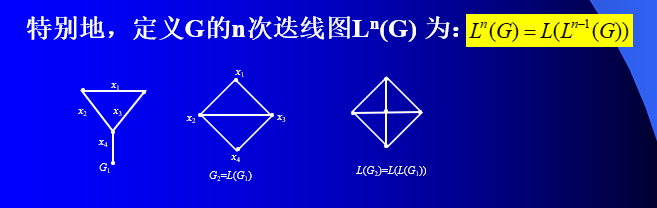#### 2、线图的性质

(1) 线图L(G)顶点数等于G的边数；若e=u v是G的边，则e作为L(G)的顶点度数为：d(e)=d(u)+d(v)-2 .

(2) 若G=(n, m), 则线图L(G) 边数为：
m ( E ( L ( G ) ) = − m + 1 2 ∑ v ∈ V ( G ) d 2 ( v ) m(E(L(G))=-m+\frac{1}{2}\sum_{v\in V(G)}d^2(v)
(3) 一个图同构于它的线图，当且仅当它是圈。

(4) 若图G和G1有同构的线图，则除了一个是K3而另一个是 K 1 , 3 K_{1,3} 外，G和G1同构。

#### 3、从线图的角度考察E图与H图的关系

定义4 S n ( G ) S_n(G) 是图G的n次细分图，是指将G的每条边中都插入n个2度顶点。又记：
L n ( G ) = L ( S n − 1 ( G ) ) L_n(G)=L(S_{n-1}(G))

定理3 (1)若G是E图，则L(G) 既是E图又是H图。

(2)若G是H图，则L(G)是H图。

定理4 一个图G 是E图的充要条件是 L 3 ( G ) L_3(G) 为H图

定理5 （Chartarand）若G 是n个点的非平凡连通图，且不是一条路，则对所有 m ≥ n − 3 m \geq n-3 L m ( G ) L^m(G) 是H图。

# 总结：常用符号

C m , n C_{m,n} 一种度极大非H图族S n ( G ) S_n(G) 是图G的n次细分图,是指将G的每条边中都插入n个2度顶点。

L ( G ) L(G) G的线图

# 总结：常用性质定理

## 1、欧拉图及其性质

• 连通图G是欧拉图当且仅当G的顶点度数为偶。

• 连通非欧拉图G存在欧拉迹当且仅当G中只有两个顶点度数为奇数。

## 2、最优欧拉环游

如果一个非负权的边赋权图G中只有两个奇度顶点u与y，求其最优欧拉环游的算法。
（1）、在u与v间求出一条最短路P；（最短路算法）
（2）、在最短路P上，给每条边添加一条平行边得G的欧拉母图G；
（3）、在G的欧拉母图G中用Fleury算法求出一条欧拉环游。

## 3、哈密尔顿图性质与判定

1、性质定理【必要条件】:若G为H图，则对V(G)的任一非空顶点子集S，有： w ( G − S ) ≤ ∣ S ∣ w(G-S)\leq | S|

2、狄拉克定理【充分条件】:对于n≧3的单图G，如果G中有： δ ( G ) ≥ n 2 \delta(G) \geq \frac{n}{2} ,则G是H图.

3、奥尔定理【充分条件】:对于n≧3的单图G，如果G中的任意两个不相邻顶点u与v，有： d ( u ) + d ( v ) ≥ n d(u) + d(v) \geq n ，那么，G是H图。

4、邦迪定理（闭包定理）【充分必要条件】:图G是H图当且仅当它的闭包是H图

5、邦迪-沙瓦达定理（度序列判定法）【充分条件】:设简单图G的度序列是(d1,d2,…,dn), 这里，d1≦d2≦…≦dn,并且n≧3.若对任意的m<n/2,或有 dm>m,或有dn-m ≧ n-m,则G是H图

7、边数判定法:设G是n阶简单图。若n≥3且KaTeX parse error: Undefined control sequence: \matrix at position 14: |E(G)>\left( \̲m̲a̲t̲r̲i̲x̲{{n-1}\\2}\righ…则G是H图；并且具有n个顶点条KaTeX parse error: Undefined control sequence: \matrix at position 8: \left( \̲m̲a̲t̲r̲i̲x̲{{n-1}\\2}\righ…边的非H图只有 C 1 , n C_{1,n} 以及 C 2 , 5 C_{2,5}

# 总结：一些结论

• 著名的彼德森图是非H图
• 若连通图G不是2-连通的，则G不是哈密尔顿图
• G为n阶简单图，若任意两个顶点存在d(u)+d(v)大于等于n-1，则该图G存在H路

欧拉图相关等价命题：

• 每个点的度为偶数
• 是连通图
• 边集可以划分为边不重的圈的并

欧拉图的相关结论：

• 一定是连通图
• 欧拉图不一定没有割点，比如8字形的图
• 欧拉图一定没有割边
• 非平凡的欧拉图中一定有圈
• 至少具有两个点的无环欧拉图一定是2边连通的

彼得森图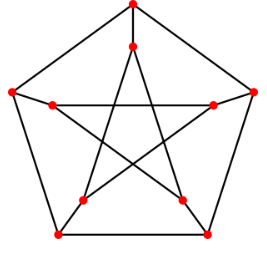，其相关结论有：

• 点连通度为3，边连通度为3
• 是一个3正则图
• 点色数为3，边色数为4
• 半径与直径均为2
• 不是H图（删去任意顶点后为H图）
• 是不可平面图
• 存在完美匹配
• 虽然该图无割边，但也不可1-因子分解（3正则图有割边，不能1-因子分解）
• 是一个1-因子和一个2-因子的并

欧拉迹相关结论：

• 连通图存在欧拉迹当且仅当G最多有两个奇度顶点
• 有向图中存在欧拉迹，当且仅当D连通且除了两个点外，每个点出度与入度相等。而这两个点中，一个点入度比出度大1，另一个点出度比入度大1

H图相关结论：（举反例想到长度为５的圈）

• 一定没有割边
• 不一定没有割点，比如H图+自环（也是H图，而自环让该点成为了割点）
• 一个简单图是H图当且仅当它的闭包是H图
• G是n≥3的简单图，若G的闭包是完全图，则G是H图
• 若G是阶数至少为3的简单图，其中任何两个不邻接的点u和v均有d(u)+d(v)≥n，则 G是H图
• 若G是阶数至少为3的简单图，若G中每个点的度d(v)≥n/2，则G是H图
• 图G的闭包是Kn，则G是H图
• G为阶数至少为3的非H的简单图，G度弱于某个Cm,n图（度极大的H图）
• H图不一定是完全图，比如长度为5的圈
• G为阶数至少为3的H简单图，若n为奇数，则G一定不是偶图
展开全文• 邦迪图论经典教材的参考答案,想学图论的较佳的书籍
• J.A. Bondy和U.S.R. Murty著的《Graph ...还记得兰州交通大学的张忠辅教授说过，国内第一届图论学会就是把大家集中起来学习邦迪的《Graph Theory with Application》，由此可见这本书对国内图论届的影响是如此之大。
• title: 图论期末考试复习 date: 2020-08-17 09:01:09 tags: 参考资料：《图论及其应用》 高等教育出版社 张先迪 / 李正良 仅用于方便复习公式查阅，公式或多有误，请以教材为准。 文章目录title: 图论期末考试...

## title: 图论期末考试复习 date: 2020-08-17 09:01:09 tags:

参考资料：《图论及其应用》 高等教育出版社 张先迪 / 李正良
仅用于方便复习公式查阅，公式或多有误，请以教材为准。

# 引言# 第一章 图的基本概念

## 图与简单图

### 图的定义及其相关概念

• 定义1：一个图是一个序偶<V,E>，记为G=(V,E),其中：
• (1) V是一个有限的非空集合，称为顶点集合,其元素称
• 为顶点或点。用|V|表示顶点数；
(2) E是由V中的点组成的无序对构成的集合，称为边集，
其元素称为边，且同一点对在E中可以重复出现多次。用
|E|表示边数。
• 相关概念
• 有限图：顶点集和边集都有限的图称为有限图。
• 平凡图与空图：只有一个顶点的图称为平凡图；只有点
没有边的图称为空图
• n阶图：顶点数为n的图，称为n阶图。
• (n, m) 图：顶点数为n的图，边数为m的图称为(n, m) 图。
• 边的重数：连接两个相同顶点的边的条数称为边的重
数；重数大于1的边称为重边。
• ：端点重合为一点的边称为环。
• 简单图：无环无重边的图称为简单图；其余的图称为
复合图。
u与v称为该边的两个端点。
• 点u与边e相关联：顶点u是边e的端点。
• 边e1与边e2相邻接：边e1与边e2有公共端点。

### 图的同构

• 定义2：设有两个图G1=(V1,E1)和G2=(V2,E2),若在其顶点
集合间存在双射，使得边之间存在如下关系：u1,v1$\in$ V1,
u2,v2$\in V 2 ， 设 u 1 ↔ u 2 ， v 1 ↔ v 2 , ; u 1 v 1 V2 ，设u1↔u2，v1↔v2,; u1v1 \in$E1 当且仅当u2v2 $\in E 2 , 且 u 1 v 1 与 u 2 v 2 的 重 数 相 同 。 称 G 1 与 G 2 同 构 ， 记 为 ： E2, 且u1v1与u2v2的重数相同。称G1与G2同构，记为： G 1 ≅ G 2 {G_1} \cong {G_2}$
• 1、图同构的两个必要条件： (1) 顶点数相同；(2) 边数相同。
• 例3 下面两图同构吗？请给出证明。
• 证明:作映射f : vi ↔ ui
• 容易证明，对 ∀ \forall vi v j $\in E ( ( a ) ) , 有 f ( v i , v j ) = u i u j E ((a)),有f (v i ,vj) = uiuj \in$E ((b))

## 完全图偶图与补图

• 完全图
• 偶图
• 完全偶图
• 简单图和补图
• 自补图
• 定义4 如果图G与其补图同构，则称G为自补图。

• 证明

## 顶点的度与图的度序列

• 定义5 G的顶点v的度d (v)是指G中与v关联的边的数目，每个环计算两次

#### 定理2 握手定理 图G= (V, E)中所有顶点的度的和等于边数m的2倍，即：• 推论1 在任何图中，奇点个数为偶数。
• 推论2 正则图的阶数和度数不同时为奇数 。
• 例3 Δ与δ是简单图G的最大度与最小度，求证：• 证明：握手定理

### 图的度序列及其性质

• 定义6 一个图G的各个点的度d1, d2,…, dn构成的非负整数组
(d1, d2,…, dn)称为G的度序列。
• 1、一个图的度序列与序列中元素排列无关；

#### 定理3 非负整数组(d1,d2,…., d n)是图的度序列的充分必要条件是序列中元素总和为偶数。

• 证明：
• 必要性： 握手定理
• 充分性： 构造法（若di为偶数，则在与之对应的点作di/2个环；对于剩下的偶数个奇数，
顶点画dj-1/2个环。该图的度序列就是已知数组。）

### 图序列及其性质

• 定义7 一个非负整数组如果是某简单图的度序列，我们称
它为可图序列，简称图序列。

#### 定理4 非负整数组是图序列的充分必要条件是：是图序列。

#### 定理5 (厄多斯1960) 非负整数组

π = ( d 1 , d 2 , ⋯   , d n ) , d 1 ≥ d 2 ≥ ⋯ ≥ d n , ∑ i = 1 n d i = 2 m \pi = ({d_1},{d_2}, \cdots ,{d_n}),{d_1} \ge {d_2} \ge \cdots \ge {d_n},\sum\limits_{i = 1}^n {{d_i} = 2m}
是图序列的充分必要条件是：• 图的频序列及其性质：
• 定义8 设n阶图G的各点的度取s个不同的非负整数
d1,d2,…, ds。又设度为di的点有bi个 (i = 1,2,…,s)，则故非整数组(b1,b2,…, bs)是n的一个划分，称为G的频序列

#### 定理6 一个简单图G的n个点的度不能互不相同.

• 注：一个简单图频序列中 至少有一个元素大于或等于2。
• 证明： 因为图G为简单图，所以：△(G)≤n-1。 鸽笼原理。

#### 定理7 一个n阶图G和它的补图有相同的频序列。

• 证明： 设图G的任一顶点v的度数为k,则该顶点在补图中的度数为n-1-k。因此：在G中有b个度数为k的顶点，则在补图中就有b个度数为n-1-k个顶点。

#### 作业 P29—P30 8, 9, 10, 11

• 9、证明：若k正则偶图具有二分类V= V1∪V2，则 | V1| = |V2|。
• 证明： 由于G为k正则偶图，所以，k |V1| =m = k|V2| >> |V1|= |V2|。
• 12、证明：若δ≥2，则G包含圈。

## 子图与图运算

### 子图的相关概念

• 子图
• 边导出子图
• 点导出子图
• 生成子图 定义3 如果图G的一个子图包含G的所有顶点，称
该子图为G的一个生成子图。

### 图运算

• 图的删点、删边运算
• 删点 在G中删去v中的顶点和G中与之关联的所有边的操作，称为删点运算
• 删边
• 注：删点要删关联的边，删边不删关联的点！
• 图的并运算 G 1 ∪ G 2 G1 \cup G2
• 点和边 均取交集
• 如果G1,G2不相交(没有公共顶点)，称它们的并为直接并，可以记为： G 1 + G 2 G1 + G2
• 图的交运算
• 点和边 均取交集
• 图的差运算
• 设G1,G2是两个图，G1与G2的差是指从G1中删去G2中的边得到的新图。记为G1-G2.
• 图的对称差运算(或环和运算)
• 设G1,G2是两个图，G1与G2的对称差定义为：• 图的联运算
• 设G1,G2是两个不相交的图，作G1+G2，并且将G1中每个顶点和G2中的每个顶点连接，这样得到的新图称为G1与G2的联图。记为 ：• 图的积图
•• 图的合成图
•• “超立方体”
•• n立方体的构造：
• n方体Q n的顶点可用一个长度为n的二进制码来表示。Q n的顶点数目正好等于2n个。
• 由n-1方体Q n-1构造Q n的方法是：将Q n-1拷贝一个。将原Q n-1每个顶点的码前再添加一个零，将拷贝得来的n-1方体每个顶点的码前面再添加一个1。然后在两个n-1方体之间连线：当且仅当两个顶点码只有一位对应位数字不同时，该两点连线。如此得到的图即为n方体

## 路与连通性

### 路与圈的相关概念

• 图中的途径
• G 的一条途径（或通道或通路）是指一个有限非空序列：
w= v0 e1 v1 e2 v2…ek vk，它的项交替地为顶点和边，使得ei的端点是vi-1和vi.(1≤i≤k).
• 途径中边数称为途径的长度；v0,vk分别称为途径的起点与终点，其余顶点称为途径的内部点。
• 图中的迹 边不重复的途径称为图的一条
• 图中的路 顶点不重复的途径称为图的一条
• 1、路是途径，也是迹，迹是途径；
• 2、起点与终点重合的途径、迹、路分别称为图的闭途径、闭迹与圈闭迹也称为回路。长度为k的圈称为k圈，k为奇数时称为奇圈，k为偶数时称为偶圈。#

### 连通性相关概念

• 两顶点的距离

• 图中顶点u与v的距离：u与v间最短路的长度称为u与v间距离。记为
d (u, v)， 如果u与v间不存在路，定义d (u, v)=∞.
• 两顶点的连通性 图G中点u与v说是连通的，如果u与v间存在途径。否则称u与v不连通。容易知道：点的连通关系是等价关系。

• 连通图与连通分支

• (1) 如果图G中任意两点是连通的，称G是连通图，否则，称G是非连通图。
• (2)非连通图中每一个极大连通部分，称为G的连通分支。G的连通分支的个数，称为G的分支数，记为 ω ( G ) \omega(G) .
• 图的直径

• 连通图G的直径定义为：• 如果G不连通，图G的直径定义为无穷大

### 偶图的判定定理

#### 定理2 一个图是偶图当且当它不包含奇圈。

• 证明
• 必要性： 一来一回
• 充分性:
• 在G中任意选取点u, 定义V的分类如下：
• X = {x | d (u, x) 是偶数，x ∈V (G)}
• Y = {y | d (u, y) 是奇数，y ∈V (G)}
• 下面证明：对X中任意两点v与w , v与w不邻接即可！• 注： P − 1 Q P^{-1}Q 为偶，如果vw邻接，则 P − 1 Q u v P^{-1}Quv 为奇圈

#### 作业 P29—P30 13, 14, 20, 22

• 13、 证明：若G是简单图且δ≥2，则G包含长至少是δ+1的圈。
• 14、G的围长是指G中最短圈的长；若G没有圈，则定义G的围长为无穷大。证明：
• （1） 围长为4的k的正则图至少有2k个顶点，且恰有2k个顶点的这样的图（在同构意义下）只有一个。
• （2） 围长为5的k正则图至少有k2+1个顶点

分析： k正则图 \delta = k = \Delta,2m=kn
围长： 用邻点集合来分析

• 20、证明：若G的直径大于3，则G的补图的直径小于3。

考虑G中任意两点u,v
- u,v 相邻
- u,v 不相邻
- 若在V(G)中任意顶点至少和u,v之一相连，>>G的直径大于3，矛盾
- 所以 存在一点w,使得uw,wv ∉ \notin E(G)

• 22．证明：若G是至少有三个点的简单连通图但不是完全图，则G有三个顶点u, v和w，使得 uv, vw∈E，而uw ∉ \notin E。

由于G是非完全连通图，所以在G中必然存在不邻接的两点## 最短路及其算法

### 最短路应用

• 状态转换问题(最少的状态转换次数)
• 例2 某两人有一只8升的酒壶装满了酒，还有两只空壶，分别为5升和3升。求最少的操作次数能均分酒。
• 例3 在一河岸有狼，羊和卷心菜。摆渡人要将它们渡过河去，由于船太小，每次只能载一样东西。由于狼羊，羊卷心菜不能单独相处。问摆渡人至少要多少次才能将其渡过河？
• 某公司在六个城市C1,C2,C3,C4,C5,C6中有分公司，从Ci到Cj的直接航程票价记在下述矩阵的(i, j)位置上，∞表示没有直接航程。制作一张任意两城市间的最便宜的路线表。#### 作业 P29—P30 16

• 1.16 a到其他所有所有的距离

最短路算法求解时,终止条件变为所有顶点被遍历到

## 图的代数表示及其特征

### 图的邻接矩阵

• 定义1 设G为n阶图，V={v1, v2, …, vn}, 邻接矩阵 A(G)=(aij),其中：• 邻接矩阵的性质
• (1)非负性与对称性。
• (2) 同一图的不同形式的邻接矩阵是相似矩阵
• (3) 如果G为简单图，则A(G)为布尔矩阵;行和(列和)等于对应顶点的度数；矩阵元素总和为图的总度数，也就是G的边数的2倍。
• G连通的充分必要条件是：A(G)不能与如下矩阵相似：• 证明：
• 必要性：vi (1≤i≤k)与vj (k+1≤i≤n)不邻接
• 充分性：设G1与G2是G的两个不连通的部分，并且设
V(G1)={v1,v2,…,vk}, V(G2)={vk+1,vk+2,…,vn}, 如果在写G的邻接矩阵时，先排V(G1)中点，再排V(G2)中点，则G的邻接矩阵形式必为：
• 这个性质说明：非连通图的邻接矩阵一定能够写成准
对角矩阵形式。

#### 定理1 设，则 a i j ( k ) a_{ij}^{(k)} 表示顶点vi到顶点vj的途径长度为k的途径条数。

• 证明： 对k作数学归纳

•• 设vm是vi到vj的途径中点，且该点和vj邻接。则vi到vj的经过vm且长度为k的途径数目应该为：• vi到vj的长度为k的途径数目为：• 推论: (1) A 2 A^2 的元素 a i i ( 2 ) aii^{(2)} vi的度数 A 3 A^3 的元素 a i i ( 3 ) aii^{(3)} 是含vi的三角形个数的2倍

### 图的关联矩阵

• 定义2 若G是(n, m) 图。定义G的关联矩阵：• 关联矩阵的性质
• (1) 关联矩阵的元素为0，1或2；
• (2) 关联矩阵的每列和为2；每行的和为对应顶点度数.

## 极图

### 邻接谱、邻接代数与图空间

• 图的邻接谱
• 定义1：图的邻接矩阵A(G)的特征值及其重数，称为G的邻接谱。
• Kn的邻接谱为：• 定义2 若两个非同构的n阶图具有相同的谱，则称它们是同谱图
• 邻接谱的两个性质
• 定理1 设单图A(G)的谱为：• 证明：• 定理2 设λ是单图G = (n, m)的任意特征值，则：• 图的邻接代数
• 定义3：设A是无环图G的邻接矩阵，则：对于矩阵的加法和数与矩阵的乘法来说作成数域C上的向量空间，称该空间为图G的邻接代数。
• 定理3：G为n阶连通无环图，则：• 图空间

### 托兰定理

• l 部图的概念与特征

• 定义4 若简单图G的点集V有一个划分：且所有的Vi非空，Vi内的点均不邻接，称G是一个l 部图。• 定理5 n阶l部图G有最多边数的充要条件是G ≌ T l , n T_{l,n}

• 度弱 设G和H是两个n阶图，称G度弱于H，如果存在双射μ：V(G)→V(H),使得：#### 定理7（Turán）若G是简单图，并且不包含 K l + 1 K_{l+1} ,则： m ( G ) ≤ m ( T l , n ) m(G) \leq m(T_{l,n})

仅当• 不含 K l + 1 K_{l+1} 的极值图是完全l几乎等部图。

#### 不含子图H( K l + 1 K_{l+1} )最多边数： m ( n , K l + 1 ) = ( l − 1 ) ( n 2 − r 2 ) / ( 2 l ) + C ( r , 2 ) m(n,K_{l+1}) = (l-1)(n^2-r^2)/(2l)+C(r,2)

• 托兰定理应用：工兵排雷问题

# 第二章 树

## 树的概念与性质

• 树的概念
• 定义1 不含圈的图称为无圈图，树是连通的无圈图。
• 定义2 称无圈图G为森林。
• 注: (1)树与森林都是单图;
• (2) 树与森林都是偶图。

#### 定理1 每棵非平凡树至少有两片树叶。

• 证明 设P=v1v2…vk是非平凡树T中一条最长路，则v1与vk在T中的邻接点只能有一个，否则，要么推出P不是最长路，要么推出T中存在圈，这都是矛盾！即说明v1与v2是树叶。

#### 定理2 图G是树当且仅当G中任意两点都被唯一的路连接。

• 证明：
• “必要性” 若不然，设P1与P2是连接u与v的两条不同的路。则由这两条路的全部或部分将构成一个圈，这与G是树相矛盾。
• “充分性” 首先，因G的任意两点均由唯一路相连，所以G是连通的。其次，若G中存在圈，则在圈中任取点u与v，可得到连接u与v的两条不同的路，与条件矛盾。

### 定理3 设T是(n, m)树，则： m = n − 1 m = n - 1

• 证明：对n作数学归纳。
• 由定理1 T中至少有两片树叶，设u是T中树叶，考虑
T1=T-u,则T1为k阶树，于是m(T1)=k-1, 得m(T)=k。

#### 定理4 每个n阶连通图的边数至少为n-1.

• 证明：
• 如果n阶连通图没有一度顶点，那么由握手定理有： m ( G ) = 1 2 ∑ v ∈ V ( G ) d ( v ) ≥ n m(G) = {1 \over 2}\sum\limits_{v \in V(G)}^{} {d(v)} \ge n
• 如果G有一度顶点。对顶点数作数学归纳。
• n=1
• n=k
• n=k+1 设u是G的一度顶点,G-u为具有k个顶点的连通图。
• 若G-u有一度顶点，则由归纳假设，其边数至少k-1,于是G的边数至少有k条；
• 若G-u没有一度顶点，则由握手定理： m ( G ) = 1 2 ∑ v ∈ V ( G ) d ( v ) ≥ n m(G) = {1 \over 2}\sum\limits_{v \in V(G)}^{} {d(v)} \ge n
• 所以G至少有k+1条边。

#### 定理5 任意树T的两个不邻接顶点之间添加一条边后，可以得到唯一圈。

• 证明： 路的唯一 到圈的唯一
• 例8 设G是树且Δ≧k,则G至少有k个一度顶点。
• 证明： 反证：握手定理+树的性质
• 例9设G是森林且恰有2k个奇数顶点，则在G中有k条边不重合的路P1, P2 ,…, Pk,使得： E ( G ) = E ( P 1 ) ∪ E ( P 2 ) ∪ ⋯ ∪ E ( P k ) E(G) = E({P_1}) \cup E({P_2}) \cup \cdots \cup E({P_k})
• 证明：对k作数学归纳。
• 当k=1时，G只有两个奇数度顶点，此时，容易证明，G是一条路；
• 设当k=t时，结论成立。令k=t+1
• 在G中一个分支中取两个一度顶点u与v，令P是连接该两个顶点的唯一路，则G-P是有2t个奇数顶点的森林，由归纳假设，它可以分解为t条边不重合的路之并，所以G可以分解为t+1条边不重合的路之并。

#### 定理6 设S=｛d1,d2,…,dn｝是n个正整数序列，它们满足：d1≧d2≧…≧dn ,∑di=2(n-1).则存在一颗树T，其度序列为S。

• 证明：对n作数学归纳。
• 当n=1和2时，结论显然。
• 假设对n=k时结论成立。设n=k+1
• 首先,序列中至少一个数为1，否则，序列和大于2k，与条件相矛盾！
• 所以，dk+1=1.我们从序列中删掉d1和dk+1,增加数
d* =d1-1放在它应该在的位置。得到序列S1.该序列含k个数，序列和为2(k-1),由归纳假设，存在树T1，它的度序列为S1.
• 现在，增加结点v，把它和T1中点d*相连得到树T。树T为所求。

## 树的中心与形心

• (1)图的顶点的离心率 e ( v ) = max ⁡ { d ( u , v ) ∣ u ∈ V ( G ) } e(v) = \max \left\{ {d(u,v)\left| {u \in V(G)} \right.} \right\}
• (2)图的半径 r ( G ) = min ⁡ { e ( v ) ∣ v ∈ V ( G ) } r(G) = \min \left\{ {e(v)\left| {v \in V(G)} \right.} \right\}
• (3)图的直径：最大离心率。
• (4)图的中心点：离心率等于半径的点。
• (5)图的中心：中心点的集合

#### 定理7 每棵树的中心由一个点或两个相邻点组成。

• 证明：对树T的阶数n作归纳证明。
• 当n=1或2时，结论显然成立。
• 设对n<k(k≧3)的树结论成立。设T是k阶树。
• 容易知道：删掉T的所有叶，得到的树T1的每个点的离心率比它们在T中离心率减少1。又因T的叶不能是中心点，所以T的中心点在T1中。这样，若点u的离心率在T中最小，则在T1中依然最小，即说明T的中心点是T1的中心点，反之亦然。
• 因为T1的阶数<k,所以，由归纳假设，T1的中心为一个点或两个相邻点组成，即证明T的中心由一个点或两个相邻点组成
• 树的形心概念与性质
• 设u是树T的任意一个顶点，树T在顶点u的分支是指包含u作为一个叶点的极大子树其分支数为顶点u的度数；树T在u点的分支中边的最大数目称为点u的权；树T中权值最小的点称为它的一个形心点。全体形心点的集合称为树T的形心。

#### 作业 P43 习题2 ： 1，2，3，4，5，6

• 2.1 证明：非平凡树的最长路的起点和终点均是 1 度的。
• 反证
• 2.2 证明：每棵恰有两个1 度顶点的树均是路。
• 反证: 握手定理+ 树的性质
• 2.3 若G 是树且最大度 >= k ，则G 至少有k个 1 度顶点。
• 反证 ： 握手定定理+树的性质
• 2.4 见例题9
• 2.5 证明：正整数序列1 2 ( , , , ) k d d  d 是一棵树的度序列当且仅当度数和为2(n-1)
• 证明：
• 必要性： m=n-1
• 充分性： 对n作数学归纳 (见定理6)
• 2.6 设T 是有k 1个顶点的任意一棵树。证明：若G 是简单图且 δ ≥ k \delta\geq k ，则G 有一个
子图同构于T 。
• 证明：对k进行归纳。
• 当k=1时，结论显然成立
• k=n 显然成立
•## 生成树

### 生成树的概念与性质

• 定义1 图G的一个生成子图T如果是树，称它为G的一棵生成树；若T为森林，称它为G的一个生成森林。
• 生成树的性质

#### 定理1 每个连通图至少包含一棵生成树。

• 证明：如果连通图G是树，则其本身是一棵生成树；若连通图G中有圈C，则去掉C中一条边后得到的图仍然是连通的，这样不断去掉G中圈，最后得到一个G的无圈连通子图T，它为G的一棵生成树
• 推论 若G是(n, m)连通图，则m≧n-1

### 生成树的计数

#### 凯莱递推计数法

• 定义2 图G的边e称为被收缩，是指删掉e后，把e的两个端点重合，如此得到的图记为G.e
• 用τ(G)表示G的生成树棵数。

#### 定理2 (Cayley) 设e是G的一条边，则有： τ ( G ) = τ ( G − e ) + τ ( G e ) \tau (G) = \tau (G - e) + \tau (Ge)

• 证明：对于G的一条边e来说，G的生成树中包含边e的棵数为τ(G.e )，而不包含e的棵数为τ (G-e).
• 例题#### 关联矩阵计数法

• 定义3 ：n×m矩阵的一个阶数为min｛n, m｝的子方阵，称为它的一个主子阵；主子阵的行列式称为主子行列式。
• 显然，当n<m时，n×m矩阵 C m n C_m^n 个主子阵。

#### 定理3 设Am是连通图G的基本关联矩阵的主子阵，则Am非奇异的充分必要条件是相应于Am的列的那些边构成G的一棵生成树。

• 该定理给出了求连通图G的所有生成树的方法：
• (1) 写出G的关联矩阵，进一步写出基本关联矩阵(选一个点，去掉该点对应的行)，记住参考点； （因为m=n-1）
• (2) 找出基本关联矩阵的非奇异主子阵，对每个这样的主子阵，画出相应的生成树。

#### 定理4 (矩阵树定理) 设G是顶点集合为V(G)=｛v1,v2,…,vn｝,的图，设A=(aij)是G的邻接矩阵，C=(cij)是n阶方阵，其中：则G的生成树棵数为C的任意一个(代数)余子式的值。

• 定理中的矩阵C又称为图的拉普拉斯矩阵，又可定义为： C = D ( G ) − A ( G ) C = D(G) - A(G) 其中，D(G)是图的度对角矩阵，即主对角元为对应顶点度数，其余元素为0。A(G)是图的邻接矩阵。
• 例4 证明τ(Kn)=nn-2(教材上定理7）

### 回路系统简介

• 定义4 连枝 树枝 设T是连通图G的一棵生成树，把属于G但不属于T的边称为G关于T的连枝，T中的边称为G关于T的树枝。
• 定义5 基本回路 设T是连通图G的一棵生成树，由G的对应于T一条连枝与T中树枝构成的唯一圈C，称为G关于T的一个基本圈或基本回路。若G是(n, m)连通图，把G对应于T的m-n+1个基本回路称为G对应于T的基本回路组。记为Cf…
• 基本回路的性质:

#### 定理4 设T是连通图G=(n, m) 的一棵生成树,C1, C2,…,Cm-n+1是G对应于T的基本回路组。定义：1.Gi=Gi , 0.Gi=Φ,Gi是G的回路。则G的回路组作成的集合对于该乘法和图的对称差运算来说作成数域F=｛0,1｝上的m-n+1维向量空间。

• 说明: 连通图G的所有回路作成子图空间的一个子空间，该空间称为回路空间或回路系统。
• 例5 求下图G的回路空间的一个基底和它的全部元素。

#### P43 习题2 ： 12, 14, 15

• 2.12 求K3,3的生成树数量
• 2.14 证
• 2.15分析：

• 选边
• 破圈法，不同的破圈方式

## 最小生成树

### 克鲁斯克尔算法

• 思想： 从G中的最小边开始，进行避圈式扩张。

• 定理1 由克鲁斯克尔算法得到的任何生成树一定是最小生成树。(证明略)

### 管梅谷的破圈法

• 破圈法求最小生成树的求解过程是：从赋权图G的任意圈开始，去掉该圈中权值最大的一条边，称为破圈。不断破圈，直到G中没有圈为止，最后剩下的G的子图为G的最小生成树。

### Prim算法

• 对于连通赋权图G的任意一个顶点u，选择与点u关联的且权值最小的边作为最小生成树的第一条边e1;
• 在接下来的边e2,e3,…,en-1 ,在与一条已经选取的边只有一个公共端点的的所有边中，选取权值最小的边。
• 反证法可以证明该算法。即证明：由Prim算法得到的生成树是最小生成树。(证明略)

### 根树简介

• 定义2：一棵树T，如果每条边都有一个方向，称这种树为有向树。对于T的顶点v来说，以点v为终点的边数称为点v的入度，以点v为起点的边数称为点v的出度。入度与出度之和称为点v的度。
• 定义3 根树：一棵非平凡的有向树T，如果恰有一个顶点的入度为0，而其余所有顶点的入度为1，这样的的有向树称为根树。其中入度为0的点称为树根，出度为0的点称为树叶，入度为1，出度大于1的点称为内点。又将内点和树根统称为分支点
• 定义4：对于根树T，顶点v到树根的距离称为点v的层数；所有顶点中的层数的最大者称为根树T的树高
• 定义5：对于根树T，若规定了每层顶点的访问次序，这样的根树称为有序树
• 注：一般次序为从左至右。有时也用边的次序代替顶点次序。
• 定义6：对于根树T，由点v及其v的后代导出的子图，称为根树的子根树。
• 定义7：对于根树T，若每个分支点至多m个儿子，称该根树为m元根树；若每个分支点恰有m个儿子，称它为完全m元树

#### 定理2 在完全m元树T中，若树叶数为t , 分支点数为i , 则： ( m − 1 ) i = t − 1 (m - 1)i = t - 1

• 证明：一方面，由树的性质得： m ( T ) = ( i + t ) − 1 ⋯ ( 1 ) m(T) = (i + t) - 1 \cdots (1)

• 另一方面，由握手定理得：

• 2 m ( T ) = t + m + ( i − 1 ) ( m + 1 ) ⋯ ( 2 ) 2m(T) = t + m + (i - 1)(m + 1) \cdots (2)

• 例5 一台计算机，它有一条加法指令，可以计算3个数的和。如果要求9个数的和，问至少执行多少次加法指令？

• 对于一棵有序树，常要转化为二元树。方法是：

• (1) 从根开始，保留每个父亲同其最左边儿子的连线，撤销与别的儿子的连线；
• (2) 兄弟间用从左至右的有向边连接；
• (3) 按如下方法确定二元树中结点的左右儿子：直接位于给定结点下面的儿子，作为左儿子，对于同一水平线上 与给定结点右邻的结点，作为右儿子，依此类推。
• 二元树的遍历问题

• 最优二元树

• 定义8 设T是一棵二元树，若对所有t片树叶赋权值wi(1≦i≦t),且权值为wi的树叶层数为L(wi),称：
W ( T ) = ∑ i = 1 t w i L ( w i ) W(T) = \sum\limits_{i = 1}^t {{w_i}} L({w_i})
为该赋权二元树的权。而在所有赋权为wi的二元树中
W(T)最小的二元树称为最优二元树。

• 哈夫曼算法：

• (1) 初始：令S=｛w1,w2,…,wt｝;
• (2) 从S中取出两个权值最小者wi与wj ,画结点vi ,带权wi,画结点vj，带权wj，画vi与vj的父亲v，连接vi与v,连接vj与v,令v带权wi + wj ;
• (3) 令S = (S-｛wi ,wj｝)∪｛wi+wj｝;
• (4) 判断S是否只含一个元素，若是，停止，否则转2).

• 2.16
• 2.17
• 2.18（未弄懂

# 第三章 图的连通度

## 割边，割点和块

### 割边及其性质

• 定义1 边e为图G的一条割边，如果 ω ( G − e ) > ω ( G ) \omega (G - e) > \omega (G)
• 注：割边又称为图的“桥”。

• 证明：
• 必要性：
• 充分性：

#### 推论1 e为连通图G的一条边，如果e含于G的某圈中，则G-e连通。

• 例1 求证: (1) 若G的每个顶点的度数均为偶数，则G没有割边; (2) 若G为k正则二部图(k≧2)，则G无割边。
• 证明：
• （1）

### 割点及其性质

• 定义2 在G中，如果E(G)可以划分为两个非空子集E1与E2,使G[E1]和G[E2]以点v为公共顶点，称v为G的一个割点
• 注： 环的点算割点

• 证明：

#### 定理3 v 是树T的顶点，则v是割点，当且仅当v是树的分支点。

• 证明：
• 例2 求证：无环非平凡连通图至少有两个非割点
• 例3 求证：恰有两个非割点的连通单图是一条路。
• 一个单图的任意生成树为路，则该图为圈或路
• 例4 求证：若v是单图G的割点，则它不是G的补图的割点。

### 块及其性质

• 定义3 没有割点的连通图称为是一个块图，简称块；G的一个子图B称为是G的一个块，如果(1), 它本身是块；(2), 若没有真包含B的G的块存在。

#### 定理5 若|V(G)|≧3,则G是块，当且仅当G无环且任意两顶点位于同一圈上。

• 证明：
• 必要性: G不能有割点，显然无环
• 证任意u,v在同一圈
• 对d(u,v)做数学归纳
• 充分性： 反证

#### 定理6 点v是图G的割点当且仅当v至少属于G的两个不同的块。

• 证明：

• 块割点树 为了直观反映图的块和割点之间的联系，引进所谓的块割点树。

• 设G是非平凡连通图。B1, B2 ,…, Bk是G的全部块，而v1,v2,…, vt是G的全部割点。构作G的块割点树 b c (G):它的顶点是G的块和割点连线只在块割点之间进行一个块和一个割点连线，当且仅当该割点是该块的一个顶点。#### P65—66 习题3 ： 1, 2, 3,5,7,8

• 3.1 证明：e是连通图G的割边当且仅当V(G)可划分为两个子集V1和V2，使对任意u∈V_1及v∈V_2, G中的路（u，v）必含e.
• 3.2
• 3.3 设G是阶大于2的连通图，证明下列命题等价：
• （1） G是块
• （2） G无环且任意一个点和任意一条边都位于同一个圈上；
• （3） G无环且任意三个不同点都位于同一条路上。

证明：
(1)>(2) : 边上插入点
(2)>(3): （存疑：如何保证任意性）G无环，且任意一点和任意一条边都位于同一个圈上，任取G的点u，边e，若u不在e上，则三个不同点位于同一个圈，即位于同一条路，如u在e上，由定理e的两点在同一个圈上，在e边插入一个点v，使得e成为2条边，由此得到新图G_1，显然G_1的是阶数大于2的块，则两条边的三个不同点在同一条路上。
(3)>(1): G连通，若G不是块，则G中存在着割点u，划分为不同的子集块V_1, V_2, V_1, V_2无环， 点u在每一条(x,y)的路上，由于x,y的任意性，则三个不同点不能位于同一条路上，则与已知矛盾，G是块。

• 3.5 证明:恰有两个非割点的连通图是路

连通 > 存在生成树
恰有两个割点 >> 生成树只有两个非割点(树叶) >> 生成树为路
任意生成树 为 路 >> 圈或路 >> 存在割点 只能是路

• 3.7 (同例题4)求证：若v是单图G的割点，则它不是G的补图的割点。
• 3.8 证明： 块的个数 ω + s u m ( b ( v ) − 1 ) \omega + sum (b(v)-1) b(v)为含v的块的个数 (未解决)

分析：

• 先证： ω = 1 \omega = 1 的时候： 数学归纳？
非割点只属于一个块

## 连通度

### 连通度的概念与性质

#### 点连通度与边连通度的概念

• 定义1 给定连通图G，设 V ′ ⊆ V ( G ) V' \subseteq V(G) ，若G -V’ 不连通，称V’为G的一个点割集，含有k个顶点的点割集称为k顶点割。G中点数最少的顶点割称为最小顶点割
• 定义2 在G中，若存在顶点割，称G的最小顶点割的顶点数称为G的点连通度；否则称n-1为其点连通度。G的点连通度记为k(G), 简记为k。若G不连通，k(G)=0。
• 定义3 在G中，最小边割集所含边数称为G的边连通度。边连通度记为λ(G) 。若G不连通或G是平凡图，则定义λ(G) =0
• 定义4 在G中，若k (G)≧ k, 称G是k连通的若λ(G)≧k，称G是k边连通的

• 证明：

#### 定理2 设G是**(n, m)连通图**，则： k ( G ) ≤ ⌊ 2 m n ⌋ k(G) \le \left\lfloor {{{2m} \over n}} \right\rfloor

• 证明： 握手定理 + 惠特尼定理

#### 哈拉里图:涉及可靠性通信网络构建

• 1962年，数学家哈拉里构造了连通度是k,边数为$m = \left\lfloor {{{nk} \over 2}} \right\rfloor$
的图Hk, n ,称为哈拉里图。
• 哈拉里构图
• H2r,n
• E ( H ) = { i j ∣ ∣ i − j ∣ ≤ r ( n ) ( 取 模 n 的 加 法 ) . } E(H) = \left\{ {ij\left| {\left| {i - j} \right| \le r(n)} \right(取模n的加法).} \right\}
• H2r+1,n (n为偶数)
• 先作H2r,n, 然后对1≦i≦n/2,i与i+n/2连线。
• H2r+1,n (n为奇数)
• 先作H2r,n, 然后对1≦i≦(n-1)/2,i与i+(n+1)/2连线。同时，0分别与(n-1)/2和(n+1)/2连线。

• 证明：

#### 定理4 设G是(n, m)单图，若对任意正整数k ,有： δ ( G ) ≥ n + k − 2 2 \delta (G) \ge {{n + k - 2} \over 2} 则G是k连通的。

• 证明：证明：任意删去k-1个顶点，记所得之图为H，则： δ ( H ) ≥ δ ( G ) − ( k − 1 ) ≥ n + k − 2 2 − k + 1 = n − k 2 \delta (H) \ge \delta (G) - (k - 1) \ge {{n + k - 2} \over 2} - k + 1 = {{n - k} \over 2}
• 由于δ(H)是整数，故： δ ( H ) ≥ ⌈ n − k 2 ⌉ = ⌊ n − k + 1 2 ⌋ \delta (H) \ge \left\lceil {{{n - k} \over 2}} \right\rceil = \left\lfloor {{{n - k + 1} \over 2}} \right\rfloor

• 证明：

#### P66—67 习题3 ： 1 2, 13, 14, 20

• 3.1
• 3.2
• 3.13 举例
• 3.14
• 3.20 证： n阶简单图 δ ≥ n − 1 \delta \geq n-1 k = δ k = \delta

### 敏格尔定理

• 定义1 设u与v是图G的两个不同顶点，S表示G的一个顶点子集或边子集，如果u与v不在G-S的同一分支上，称S分离u和v。

#### 定理2 (惠特尼1932) 一个非平凡的图G是k (k≧2)连通的，当且仅当G的任意两个顶点u与v间，至少存在k条内点不交的(u ,v)路。

• 例1 设G是k连通图，S是由G中任意k个顶点构成的集合。若图H是由G通过添加一个新点w以及连接w到S中所有顶点得到的新图，求证：H是k连通的。

#### 定理3 (惠特尼1932) 一个非平凡的图G是k (k≧2)边连通的，当且仅当G的任意两个顶点间至少存在k条边不重的(u ,v)路。

• 证明：

• 必要性：考虑任意两点 u,v
• 讨论 相邻 与 不相邻
• 充分性：
• 例1 设G是k连通图，S是由G中任意k个顶点构成的集合。若图H是由G通过添加一个新点w以及连接w到S中所有顶点得到的新图，求证：H是k连通的。

#### 推论 对于一个阶至少为3的无环图G，下面三个命题等价。

• (1) G是2连通的；
• (2) G中任意两点位于同一个圈上；
• (3) G无孤立点，且任意两条边在同一个圈上。
• 证明：

(1)→(2)
G是2连通的,则G的任意两个顶点间存在两条内点不交路P1与P2,显然这两条路构成包含该两个顶点的圈。
(2)→(3)
G无孤立点显然。设e1与e2是G的任意两条边，在e1与e2上分别添加两点u与v得图H，则H是2连通的，由(1)→(2)，H的任意两个顶点在同一个圈上，即u与v在同一个圈上，也即e1与e2在同一个圈上。
(3)→(1)
设u与v是无环图G的任意两个不相邻顶点，由于G无孤立点，所以可设e1,e2分别与u, v相关联。由(3),e1,e2在同一个圈上，所以u与v在同一个圈上，因此分离u与v至少要去掉两个顶点，即证明G是2连通的。

# 第一次上交作业

• 习题1 ： 4，5, 11，12, 17，18.
• 习题2 ： 1，9, 16.
• 习题3 ： 1，3, 7, 12，13.

# 第四章 欧拉图与Hamilton图

## 欧拉图及其性质

• 基本概念
• 定义一（欧拉图与欧拉回路） 对于连通图G，如果G中存在经过每条边的闭迹，则称G为欧拉图，简称G为E图。欧拉闭迹又称为欧拉环游，或欧拉回路

### 欧拉图的性质

#### 定理一 下列陈述对于非平凡连通图G是等价的

1. G是欧拉图
2. G的顶点度数为偶数
3. G的边集合能划分为圈
• 定理一 证明:
• 1 >> 2: 一进一出
• 2 >> 2: 减圈法
• 3 >> 1: 拼圈法

#### 例题：

• 例题1 证明： 欧拉图 G G 与欧拉图 H H 乘积 G × H G \times H 仍然是欧拉图.
• 先证明d((u,v)) = d(u) + d(v) [邻点 ( u , w ) (u,w) w w d ( u ) d(u) 种，邻点 u ( x , v ) u(x,v) , x x d ( v ) d(v) 种]
• 证明 G × H G\times H 是连通的 (u1,v1)与(u2,v2) 连通
（ u 1 , v 1 ） > > ( u 1 , v 2 ) > > ( u 2 , v 2 ) （u1,v1）>> (u1,v2) >> (u2,v2)
• 一笔画问题： 欧拉迹存在问题
• 几笔画问题： 添加几笔成为欧拉图

#### 其他性质：

• 欧拉图不存在割边
• 欧拉图举例
• 完全图 K n K_n ，当n为奇时为欧拉图
• n立方体 Q n Q_n ,当n为偶数为欧拉图
• 完全二部图 K a , b K_{a,b} , a , b a,b 均为偶数时为欧拉图
• 例题2若G是非平凡的欧拉图，则G的每个块也是欧拉图
• 证明： 对于任一块，欧拉回路跨越两个块，必然经过割点，按割点分割的欧拉回路，是每个块的欧拉回路。
• 例题 3 设G是非平凡的欧拉图，且v∈V(G)。证明：G的每条以v为起点的迹都能扩展成G的欧拉回路 当且仅当 G‒v是森林
• 必要性： 非B >> 非A:• 充分性：### Fleury(夫勒里)算法 (求一条具体欧拉环游的方法)

• 基本思想：尽可能避割边行走
• 算法：
• 任意选择一个顶点 v 0 v_0 ,置 w 0 = v 0 w_0 = v_0
• 假设迹 w i = v 0 e 1 v 1 . . . e i v i w_i = v_0e_1v_1...e_iv_i 已经选定，按一下要求从剩余边集合种选取下一条边 e i + 1 e_i+1 :
• e i + 1 e_i+1 v i v_i 相关联
• 除非没有的边可选择，否则 e i + 1 e_i+1 不能是 G i = G − e 1 , . . . , e i G_i = G - {e_1,...,e_i} 的割边
• 迭代第二步，直至无边可选
• 例题4 证明 G G 2 k > 0 2k>0 个奇数顶点，则存在k条边不重的迹 Q 1 , Q 2 , … , Q k Q1,Q2,…,Qk ，使得： E ( G ) = E ( Q 1 ) ∪ E ( Q 2 ) ∪ . . . ∪ E ( Q k ) E(G) = E(Q_1) \cup E(Q_2) \cup ... \cup E(Q_k)
• 证明： ( v i , v i + k v_i,v_{i+k} 间)加边 >> 欧拉图 >> 圈的集合 >> 去边 >> 迹的集合

## 中国邮路问题

• 问题：每条街道至少走一次,如何用最少路程，回到邮局。

#### 求最优环游：

• 欧拉图， 最优环游为欧拉回路
• 对一般图，解法： 添加重复边 以使得 G 成为欧拉图 G*，并使得添加的重复边的边权之和为最小，再求G* 的欧拉回路：
• 用每条边最多添一次的方法任意添一些重复边使图G成为一个欧拉多重图G′。
• 考查G′的圈，若存在圈C，其中重复边的总权值大于该圈权值的一半，则在圈C上交换重复边和不重复边得到一个新的欧拉多重图。重复这个过程，直到得到一个图G*，使得图G*中每个圈上重复边的总权值不大于该圈权值的一半。
• 用Fleury算法求G*的Euler回路。

#### 定理2（管梅谷） 若W是包含图G的每条边至少一次的闭途径，则W具有最小权值当且仅当下列两个条件被满足：

• (1) G的每条边在W中最多重复一次;
• (2) 对于G的每个圈上的边来说，在W中重复的边的总权值不超过该圈非重复边总权值。
• 定理2 证明
• 必要性：(奇度点对之间的路上的边改为2重边) >> 欧拉图G1 >> 删去重数>2的边 >> 欧拉图G2 >> 假定不满足（1）（2） 可得更优 w。（没看太懂）
• 充分性：略
• 非欧拉图，求最优环游
• 奇度点配对
• 奇度间找条路 路上添加重复边
• 按照定理2修改 修改到最优：
• 考查G′的圈，若存在圈C，其中重复边的总权值大于该圈权值的一半，则在圈C上交换重复边和不重复边得到一个新的欧拉多重图。重复这个过程，直到得到一个图G*，使得图G*中每个圈上重复边的总权值不大于该圈权值的一半。
• G ∗ G^* 的欧拉环游
• 例题5 求最优欧拉环游
•• 例6 如果一个非负权的边赋权图G中只有两个奇度顶点u与v,设计一个求其最优欧拉环游的算法。
• 算法设计：
• (1)、 在u与v间求出一条最短路P; (最短路算法)
• (2)、 在最短路P上，给每条边添加一条平行边得G的欧拉母图G*;
• (3)、 在G的欧拉母图G* 中用Fleury算法求出一条欧拉环游。
• 证明：重复边的权值和 ≥ 任意路 ≥ 最短路

## Hamilton图

### 哈密尔顿图的概念

• 定义
• 经过图中每个点的路称为Hamilton路，简称H路。
• 经过图中每个点的圈称为Hamilton圈，简称H圈。
• 存在Hamilton圈的图称为Hamilton图，简称H图。
• 思考
• Hamilton图举例
• 正十二面体图
• n > = 3 n>=3 的完全图 K n K_n
• n > = 2 n>=2 ,n立方体 Q n Q_n
• 完全二部图 K a , b K_{a,b} a = b > = 2 a=b>=2
• 是否存在一个具有奇数个顶点的连通图既是二部图，又是Hamilton图
• 不存在，否则二部图种出现了奇圈
• 二部图G是Hamilton图（二部划分为X,Y） 须满足 |X|=|Y|(必要条件)
• 例题1 若G1和G2是H图，则G1×G2是H图。
••### 性质与判定

#### 定理1（必要条件） 若G是H图，则对于V的每个非空真子集S，均有 ω ( G − S ) ≤ ∣ S ∣ ω(G-S)≤|S| 。

• 证明： ω ( G − S ) ≤ ω ( C − S ) ≤ ∣ S ∣ ω(G-S)≤ω(C-S)≤|S| 其中 C C G G G G

• 例题2 **（未看）**彼得森图不是H图，但满足定理中的条件

• 例题3 证明：若连通图不是2-连通的，则G不是Hamilton图

• 存在割点， ω ( G − v ) ≥ 2 > 1 = ∣ v ∣ ω(G-v)≥2>1=|{v}| 不满足必要条件
• 推论 Hamilton图一定不存在割点

#### 定理2 若图G包含哈密尔顿路，则对V(G)的每个真子集S， ω ( G − S ) ≤ ∣ S ∣ + 1 。 ω(G-S) ≤ |S|+1。

• 证明： ω ( G − S ) ≤ ω ( P − S ) ≤ ∣ S ∣ + 1 。 ω(G-S) ≤ ω(P-S) ≤ |S|+1。
• 例题4 若图G是哈密尔顿图且不是圈，则G至少包含2个度数不小于3的顶点。
• 证明：反证 ： 若只有一个度不小于3的顶点，则该点为割点

#### 定理3（充分条件）(Dirac 1952) 对于n≥3的简单图G，如果G中有： δ ( G ) ≥ n / 2 \delta (G) \geq n/2 则G是H图

• 证明：（反证法）
• 极大非H简单图 G ′ G' ：任意添加边uv都成为H图
• G ′ G' 的H路 P = v 1 v 2 . . . v n P = v_1v_2...v_n
• S = { v i ∣ v 1 v i + 1 ∈ E ( G ) } S=\{v_i|v1v_{i+1}\in E(G)\} T = { v i ∣ v n v i ∈ E ( G ) } T=\{v_i|v_nv_i\in E(G)\}
• S ∩ T = ∅ S\cap T = \empty 否则存在Hamilton圈
• $d(v_1)+d(v_n) = |S| +|T| < n 则 与 则与 \delta (G) \le n/2$ 矛盾

#### 定理4（充分条件）(Ore 1962) 对于n≥3的简单图G，如果G中的任意两个不相邻顶点u与v，有： d ( u ) + d ( v ) ≥ n d(u)+d(v)\geq n 则G是H图

• 注：证明与定理3一致，该定理的条件是紧的。

#### 闭图与闭包基本概念

• 闭图定义 在n阶简单图G中，若对d(u)+d(v)≥n的任何一对点u和v都是相邻的，则称G是闭图
• 定理 若G1和G2是同一个点集V的两个闭图，则 G = G 1 ∩ G 2 G=G1∩G2 是闭图。
• 证明： d G ( u ) + d G ( v ) > = n d_{G}(u)+d_{G}(v)>=n d G 1 ( u ) + d G 1 ( v ) > = n d_{G_1}(u)+d_{G_1}(v)>=n + d G 2 ( u ) + d G 2 ( v ) > = n d_{G_2}(u)+d_{G_2}(v)>=n ，容易知道u,v在G1,G2邻接所以在G中也邻接
• 闭包定义 若一个与G 有相同点集的闭图 Ĝ，使 G ⊂ G ^ G \subset Ĝ ，且对异于Ĝ的任何图H，若有 G ⊂ H ⊂ G ^ G \subset H \subset Ĝ ，则H不是闭图，则称Ĝ是G的闭包。（G的闭包是包含G的极小闭图
• 图G的闭包唯一
• 对于单图G，如果G中有两个不相邻顶点u与v，满足： d ( u ) + d ( v ) ≥ n d(u)+d(v)≥n ，那么G是H图当且仅当G + u v是H图 。
• 闭包的构造： 如果G本身是闭图，则其闭包是它本身；如果G不是闭图，则由定义可以通过在度和大于等于n的不相邻顶点对间加边来构造G的闭图。

#### 邦迪——闭包定理（充要条件） 图G是H图当且仅当它的闭包是H图。

• 推论1： 设G是n≧3的单图，若G的闭包是完全图，则G是H图。
• 推论2： 设G是n≧3的单图。若δ(G)≧n/2,则G是H图 (Dirac定理)。
• 推论3： 若对于G中任意不相邻顶点u与v，都有d(u)+d(v)≧n,则G是H图.(Ore定理)

## 非哈密尔顿图与TSP问题

### 非Hamilton图特征

• 定义1 图G称为度极大非H图，如果它的度不弱于其它非H图。
• 定义2 对于 1 ≦ m < n / 2 1≦ m <n/2 , C m , n C_{m,n} 图定义为： C m , n = K m ∨ ( K ‾ m + K n − 2 m ) C_{m,n} = K_m \vee (\overline K_m + K_{n-2m})
••#### 引理1 对于1≦m <n/2 的图 C m , n C_{m,n} 是非H图。

• 证明： 取 S = K m S = {K_m} , w ( G − S ) = m + 1 > m = ∣ S ∣ w(G-S)=m+1>m=|S| (不符合H图的必要条件)

#### 定理1 (Chvátal,1972)若G是n≧3的非H单图，则G度弱于某个 C m , n C_{m,n} 图。

• 证明：#### 推论1 n(≥3)阶单图若度优于 C m , n C_{m,n} 图族中所有图，则G是H图。• 例1(证明题) 设G是度序列为(d1,d2,…,dn)的非平凡单图，且d1≦d2≦…≦dn。证明：若G不存在小于(n+1)/2的正整数m，使得：dm<m且dn-m+1<n-m,则G有H路。
• 证明：( G 1 = G ∪ v G1 = G \cup v ) (G1的度序列为： (d1+1,d2+1,…,dn+1, n))
• 度序列判定G1为H图（由条件：不存在小于(n+1)/2的正整数m,使得dm+1≦m,且dn-m+1+1<n-m+1=(n+1)-m。） >> $G = G1 - v$ 存在H路
• 例2(应用题) 一只老鼠吃 3 ∗ 3 ∗ 3 3*3*3 立方体乳酪。其方法是借助于打洞通过所有的27个$111$的子立方体。如果它从一角上开始，然后依次走向未吃的立方体，问吃完时是否可以到达中心点？
• 解：H路存在性问题：
• 图建模 >> 偶图 划分为13:14
• |X| ！= |Y| (加边（起点-中心点）后)不能存在圈（ w ( G 1 − Y ) = 14 > ∣ Y ∣ = 13 w(G1-Y)=14>|Y|=13 ） >> 原图不能存在H路

### TSP问题（旅行售货员问题）

#### 边交换技术[有权完全图]（求近似最优哈密尔顿圈）

• 在赋权完全图中取一个初始H圈 C = v 1 v 2 , … , v n v 1 C = v1v2,…,vnv1
• 如果存在下图中红色边，且 w ( v i v i + 1 ) + w ( v j v j + 1 ) ≧ w ( v i v j ) + w ( v i + 1 v j + 1 ) w(v_iv_{i+1})+ w(v_jv_{j+1})≧w(v_iv_j)+ w(v_{i+1}v_{j+1}) ,则把C修改为：$C1=v1v2,…,vivj…vi+1vj+1…,vnv1$；
• 反复修改，直到不能修改为止。最后圈为近似最优哈密尔顿圈。• 例3 采用边交换技术求赋权完全图的一个近似最优H圈。

#### 赋权完全图中最优H圈下界估计

• (1) 在G中删掉一点v(任意的)得图G1;
• (2) 在图G1中求出一棵最小生成树T;
• (3) 在v的关联边中选出两条权值最小者e1与e2.
• 若H是G的最优圈，则： W ( H ) ≥ W ( T ) + W ( e 1 ) + W ( e 2 ) W(H)\geq W(T)+W(e_1)+W(e_2)

## 超Hamilton图与超可迹图

### 超H图与超可迹图

• 定义1 若图G是非H图，但对于G中任意点v,都有G-v是H图，则称G是超H图。
• 举例： 彼德森图
• 证明： 1. 证G为非H图 2. 证G-v为H图
• 定义2 若G中没有H路，但是对G中任意点v，G-v存在H路，则称G是超可迹的。
• 定理2 Thomassen图是超可迹图。
• 证明： 1. 证明G中不存在H路。
• 一些研究
• 普鲁默猜想：每个2连通图的平方是H图。
• 图的平方 原图 G G 中距离小于等于2的点 在 G 2 G^2 中邻接

### E图与H图的关系

#### 线图

• 定义3 设G是图，G的线图L(G)定义为： V ( L ( G ) ) = E ( G ) , ( e 1 , e 2 ∈ E ( L ( G ) ) ) ↔ 在 G 中 有 ： e 1 , e 2 邻 接 V(L(G))=E(G),(e_1,e_2\in E(L(G))) \leftrightarrow在G中有：e_1,e_2邻接
• 特别地，定义G的n次迭线图Ln(G) 为： L n ( G ) = L ( L n − 1 ( G ) ) L^n(G) = L(L^{n-1}(G))• 线图的性质
• (1) 线图L(G)顶点数等于G的边数；若e=u v是G的边，则e作为L(G)的顶点度数为： d ( e ) = d ( u ) + d ( v ) − 2 d(e)=d(u)+d(v)-2 .
• (2) 若G=(n, m), 则线图L(G) 边数为：$m(E(L(G)) = - m + \frac{1}{2}\sum\limits_{v \in V(G)}^{} {{d^2}(v)}$
• (3) 一个图同构于它的线图，当且仅当它是圈。
• (4) 若图G和G1有同构的线图，则除了一个是K3而另一个是K1,3外，G和G1同构。(证明比较复杂)
• 从线图考察E图与H图
• 定义4 称Sn是图G的n次细分图，是指将G的每条边中都插入n个2度顶点。
• L n ( G ) = L ( S n − 1 ( G ) ) {L_n}(G) = L({S_{n - 1}}(G))

### 习题4 ： 1, 2, 3, 7, 8, 9

• 习题4 ： 1, 3， 4, 7，8， 11， 15.
• 4.1 一笔画问题： 是否存在欧拉迹，即 最多只能有两个奇度顶点
• 4.2 几笔画问题：奇点数除以二便可算出此图需几笔画成
• 4.3 画图举例 —— Hamilton圈与Euler闭迹：
• 4.4 设n阶无向简单图G有m条边。证明：若m≥(n-1 2) +2，则G是哈密尔顿图。
• 证明：同第二次作业3
• 托兰定理
• 4.7 证明：若G没有奇点，则存在边不重的圈C1, C2,…, Cm，使得，E(G) = E(C1)∪E(C2)∪…∪E(Cm)。
• 去圈法
• 4.8 证明：若G有2k＞0个奇点，则存在k条边不重的迹Q1, Q2,…, Qk，使得，E(G) = E(Q1)∪E(Q2)∪…∪E(Qk)。：
• 加边 >> 欧拉图 >> 圈集合 >> 去边 >> 迹集合
• 4.9 同例题3
• 4.11 证明 定理2 若图G包含哈密尔顿路，则对V(G)的每个真子集S， ω ( G − S ) ≤ ∣ S ∣ + 1 。 ω(G-S) ≤ |S|+1。
• 4.15 同第二次作业题9

# 第五章 匹配

## 偶图的匹配问题

### 图的匹配与贝尔热定理

#### 偶图

• 定义：所谓具有二分类（X, Y）的偶图（或二部图）是指一个图，它的点集可以分解为两个(非空)子集X和Y，使得每条边的一个端点在X中，另一个端点在Y中.
• 性质
• 偶图不能有环，偶图可以有重边；
• 【判定定理】 图G是偶图当且仅当G不含奇圈。
• k-正则偶图
• k-正则偶图的两个顶点子集包含顶点个数相等。
• 对称差运算

#### 图的匹配

• 匹配 M— 如果M是图G的边子集(不含环），且M中的任意两条边没有共同顶点，则称M是G的一个匹配或对集或边独立集
• 饱和点 如果G中顶点v是G的匹配 M中某条边的端点，称它为M饱和点，否则为M非饱和点。
• 最大匹配M — 如果M是图G的包含边数最多的匹配，称M是G的一个最大匹配。特别是，若最大匹配饱和了G的所有顶点，称它为G的一个完美匹配。
• 区分
• 一个图G不一定存在完美匹配；
• 一个图G的完美匹配若存在，不一定唯一；
• 一个图G的最大匹配不一定唯一。
• M交错路与M可扩路— 如果M是图G的匹配，G中一条由M中的边和非M中的边交错形成的路，称为G中的一条M交错路。特别地，若M交错路的起点与终点是M非饱和点称这种M交错路为M可扩路

#### 定理1 最大匹配判定 (贝尔热，1957) G的匹配M是最大匹配，当且仅当G不包含M可扩路。

• 证明：
• 必要性：非B >> 非A ， 若存在M可扩路，可得更多边的匹配
• 充分性：### 偶图的匹配与覆盖

• 问题引出： 两个集合，如何匹配？

#### 定理2 (Hall定理）设G=(X, Y)是偶图，则G存在饱和X每个顶点的匹配(X选Y)的充要条件是： ∀ S ⊆ X , ∣ N ( S ) ∣ ≥ ∣ S ∣ ⋯ ( ∗ ) \forall S \subseteq X,\left| {N(S)} \right| \ge \left| S \right| \cdots (*)

• N(S)为S的邻接顶点的集合
• 证明：
• 必要性：X的每个顶点，在Y中至少有一个邻接点(A>>B)
• 充分性：（反证）假设B下有非A,然后退出非B 矛盾: 存在不饱和X的顶点u，
• 设Z为关联u的M交错路， S = X ∩ Z , T = Z ∩ Y S=X∩Z , T=Z∩Y ,
• S-｛u｝中点与T中点在M*下配对,$|N(S)| = |T| = |S| -1< |S|$
• Hall定理也可表述为：设G=(X,Y)是偶图，如果存在X的一个子集S,使得|N(S)| < |S| ，那么G中不存在由X到Y的匹配。
• 又称“婚姻定理” ：在一个由r个女人和s个男人构成的人群中，1≦r≦s。在熟识的男女之间可能出现r对婚姻的充分必要条件是，对每个整数k(1≦k≦r),任意k个女人共认识至少k个男人。(女挑选男)
• 推论 若G是k (k>0)正则偶图，则G存在完美匹配
• 证明： k ∣ X ∣ = k ∣ Y ∣ → ∣ X ∣ = ∣ Y ∣ ； k|X|=k|Y| \rightarrow |X| = |Y|； 对于X的任一非空子集S, 设E1与E2分别是与S和N(S)关联的边集，显然有 E 1 ⊆ E 2 {E_1} \subseteq {E_2} ∣ E 1 ∣ = k ∣ S ∣ ≤ ∣ E 2 ∣ = k ∣ N ( S ) ∣ \left| {{E_1}} \right| = k\left| S \right| \le \left| {{E_2}} \right| = k\left| {N(S)} \right|
• 例题2
• (1) 证明：每个k方体都有完美匹配(k大于等于2)
• 证法一：按坐标之和 的 奇 和 偶 两部分 [k方体有 2 k 2^k 个顶点,每个顶点可以用长度为k的二进制码来表示，两个顶点连线当且仅当代表两个顶点的二进制码只有一位坐标不同。] >> k正则偶图 >> 存在完美匹配
• 证法二：构造法（举例法）：
• 一些结论： k方体是k正则偶图。
• (2) 求 K 2 n K_{2n} K n , n K_{n,n} 中不同的完美匹配的个数。
• K 2 n = ( 2 n − 1 ) K 2 n − 2 K_{2n} = (2n-1)K_{2n-2} >> ( 2 n − 1 ) ! ! (2n-1)!!
• K n , n = n ∗ K n − 1 , n − 1 K_{n,n} = n * K_{n-1,n-1} >> n ! n!
• 例3 证明树至多存在一个完美匹配。
• (反证+对称差)假设存在连个匹配 M 1 , M 2 M1,M2 , M 1 Δ M 2 ≠ Φ M1ΔM2≠Φ T [ M 1 Δ M 2 ] T[M1ΔM2] 每个非空部分顶点度数为2（ ∣ M 1 ∣ = ∣ M 2 ∣ |M1|=|M2| ），即它存在圈，矛盾。

#### 点覆盖与哥尼定理

• 点覆盖的概念与性质
• 定义1 图的点覆盖 G的一个顶点子集K称为G的一个点覆盖，如果G的每条边都至少有一个端点在K中。G的一个包含点数最少的点覆盖称为G的最小点覆盖，其包含的点数称为G的覆盖数，记为α(G).

#### 定理2（点覆盖与边匹配互为上下界） 设M是G的匹配，K是G的覆盖，若|M|=|K|,则M是最大匹配，而G是最小覆盖。

- 证明：由匹配和覆盖定义有：|M*|≦|K*|。（M每条边取点,得到的点集 不一定能点覆盖（少于最小点覆盖））


#### 哥尼定理(哥尼，1931) 在偶图中，最大匹配的边数等于最小覆盖的顶点数。

• 证明：反证法
M*是偶图G的最大匹配。U表示X中M*非饱和点集。Z表示由M*交错路连到U的顶点的所有路上的点作成的集合。且令S=Z∩X, T=Z∩Y。
最小点覆盖 K ∗ = ( X − S ) ∪ T K* =(X-S)∪T
最大边覆盖 M ∗ 为 红 边 集 合 M*为红边集合
可证[ K ∗ = M ∗ K*= M* ]（M上的每条边选一个不饱和顶点）• 例4 矩阵的一行或一列称为矩阵的一条线。证明：布尔矩阵中，包含了所有“1”的线的最少数目，等于具有性质“任意两个1都不在同一条线上的1的最大数目”。
• 分析： 布尔矩阵 >> 行，列分为两部分(X表示行点集合，Y表示列点集合) (0/1表示是否有边)>> 偶图 >> 哥尼定理 >> 证之

## 图的因子分解

### 托特定理(图的完美匹配存在定理)

#### 托特定理 (托特定理，1947) 图G有完美匹配当且仅当对V的任意非空真子集S, 有： o ( G − S ) ≤ ∣ S ∣ o(G - S) \le \left| S \right|

• 注： o ( G − S ) ≤ ∣ S ∣ o(G - S) \le \left| S \right| 表示奇分支数目.(奇分支：顶点数为奇数)
• 例1 证明：一棵树G有完美匹配当且仅当对所有顶点v ∈V(G),有：o (G - v)=1。
• 证明：
• 必要性：（A >> B） 完美匹配 >> 托特定理 >> o(G - v)≤1;完美匹配 >> G 为偶阶树 >> o(G-v)≥1
• 充分性： （B>>A） 对所有顶点v ∈V(G),有：o (G - v)=1 >> 与奇分支关联的边选为匹配边(M=｛e(v):它是由v连到G-v的奇分支的边，v ∈V(G) ｝)

#### 推论 (彼得森定理)没有割边的3正则图存在完美匹配。

• 证明：
• 假设设S为任意分支，mi为S与第i个奇分支连接的边数目（0<i<=k）
• (mi = Gi在G中总度数 - Gi总度数) m i = 3 ∣ V ( G i ) ∣ − 2 ∣ E ( G i ) ∣ {m_i} = 3\left| {V({G_i})} \right| - 2\left| {E({G_i})} \right| >> m i m_i 为奇数
• 无割边 >> m i ≥ 3 m_i \geq 3 则 $k \le {1 \over 3}\sum\limits_{i = 1}^k {{m_i}}$
• 对于S有， ${1 \over 3}\sum\limits_{i = 1}^k {{m_i}} \le {1 \over 3}\sum\limits_{v \in S}^{} {d(v)}$
• 于是 o ( G − S ) = k ≤ 1 3 ∑ i = 1 k m i ≤ 1 3 ∑ v ∈ S d ( v ) ≤ 1 3 ⋅ 3 ∣ S ∣ = ∣ S ∣ o(G - S) = k \le {1 \over 3}\sum\limits_{i = 1}^k {{m_i}} \le {1 \over 3}\sum\limits_{v \in S}^{} {d(v)} \le {1 \over 3} \cdot 3\left| S \right| = \left| S \right|
• 利用托特定理得证

### 图的一因子分解

#### 基本概念

• 所谓一个图G的因子Gi，是指至少包含G的一条边的生成子图
• 所谓一个图G的因子分解，是指把图G分解为若干个边不重的因子之并
• 所谓一个图G的n因子，是指图G的n度正则因子
• 如果一个图G能够分解为若干n因子之并，称G是可n因子分解的
• 问题：
• 能否分解 如何分解
• 图的一因子分解
• 一因子实际上就是图的一个完美匹配的导出子图。一个图能够作一因子分解，也就是它能够分解为若干边不重的完美匹配的导出子图之并。

#### 定理1 K 2 n K_{2n} 可一因子分解。

• 证明：(给出分解方法) 轮换法 2n-1个边不重的一因子（每个拥有n条边）
• 例2 证明：每个k (k>0)正则偶图G是一可因子分解的。
• 证明： G存在完美匹配 >> G存在至少一个一因子 >> 设Q为G的一个一因子，G-Q仍位正则偶图 >> 递推

#### 定理2 具有H圈的三正则图可一因子分解。

• 证明: 抽取H圈，剩下的边构成一个一因子 >> 三正则图（奇度点数目为偶数（握手定理）），H圈为偶圈，可分解为两个一因子。

#### 定理3 若三正则图有割边，则它不能一因子分解。

• 反证 假设能分解，有三个一因子，任意减去一个一因子，剩下的必然为圈之并（均为偶点，存在欧拉回路），即不能有割边

### 图的二因子分解

• 如果一个图可以分解为若干2度正则因子之并，称G可以2因子分解
• 注意：G的一个H圈肯定是G的一个2因子，但是G的一个2因子不一定是G的H圈。2因子可以不连通。

#### 定理4 K 2 n + 1 K_{2n+1} 可2因子分解

• 证明：(给出分解方法)
• 顶点集 V ( K 2 n + 1 ) = { v 1 , v 2 , ⋯   , v 2 n + 1 } V({K_{2n + 1}}) = \left\{ {{v_1},{v_2}, \cdots ,{v_{2n + 1}}} \right\}
• 作路(0<i<=n,下标需要mod2n,如n=3时 P 1 = v 1 v 6 v 2 v 5 v 3 v 4 {P_1} = {v_1}{v_6}{v_2}{v_5}{v_3}{v_4} ) P i = v i v i − 1 v i + 1 v i − 2 v i + 2 v i − 3 ⋯ v i − n v i + n {P_i} = {v_i}{v_{i - 1}}{v_{i + 1}}{v_{i - 2}}{v_{i + 2}}{v_{i - 3}} \cdots {v_{i - n}}{v_{i + n}}
• 生成圈Hi为v2n+1与Pi的两个端点连线。

#### 定理5 K 2 n K_{2n} 可分解为一个1因子和n-1个2因子之和。

• 证明：给出分解方法：作n-1条路 P i = v i v i − 1 v i + 1 v i − 2 v i + 2 v i − 3 ⋯ v i − n − 1 v i + n − 1 {P_i} = {v_i}{v_{i - 1}}{v_{i + 1}}{v_{i - 2}}{v_{i + 2}}{v_{i - 3}} \cdots {v_{i - n - 1}}{v_{i + n - 1}}
• 下表按模2n-1计算，然后把v2n和Pi的两个端点连接，可以得到n-1个2因子，剩余为一因子。

#### 定理6 每个没有割边的3正则图是一个1因子和1个2因子之和。

• 证明：没有割边的三正则图 存在完美匹配M, 显然G-M为2-因子

### 图的森林因子分解

• 把一个图分解为若干边不重的森林因子的和，称为图的森林因子分解
• 荫度 图G分解为边不重的森林因子的最少数目问题，称这个最少数目为G的荫度，记为σ(G)
• 定理8 图G的荫度为：$\sigma (G) = \mathop {\max }\limits_s \left\lceil {{{{m_s}} \over {s - 1}}} \right\rceil$ 其中s是G的子图Hs的顶点数，而： m s = max ⁡ s { E ( H s ) } {m_s} = \mathop {\max }\limits_s \left\{ {E({H_s})} \right\}
• 定理9 完全图的荫度 σ ( K n ) = ⌈ n 2 ⌉ \sigma ({K_n}) = \left\lceil {{n \over 2}} \right\rceil 完全偶图的荫度 σ ( K r , s ) = ⌈ r s r + s − 1 ⌉ \sigma ({K_{r,s}}) = \left\lceil {{{rs} \over {r + s - 1}}} \right\rceil

#### 完全图的森林因子分解

• 对于K2n，将其分解为n条路Pi = vivi-1vi+1vi-2vi+2…vi-nvi+n,脚标按模2n计算。
• 对于K2n+1，先作n条路Pi = vivi-1vi+1vi-2vi+2…vi-nvi+n,脚标按模2n计算。在每条路外添上点v2n+1的n个森林因子；然后，v2n+1与v1,v2,…,v2n分别相连接得一星图，这是G的最后一个森林因子。（n条路 + 星图）
• 例8 证明：若n为偶数，且δ(G)≥n/2+1 ,则n阶单图G有3因子。
• 证明： 存在H圈 >> n为偶数，H圈可分解出一个一因子Q >> G-Q 满足δ(G)≥n/2 >> G-Q仍
存在H圈C >> C ∪ Q C \cup Q 为一个三因子

### 作业题

P117—118 习题4 ： 3, 4, 5，6，7，8，9
P97—99 习题4 ： 1, 3， 4, 7，8， 11， 15.
P117—118 习题5 ： 1, 2, 3, 4，10， 11， 12, 19.

## 匈牙利算法与最优匹配算法

### 匈牙利算法（偶图中寻找完美匹配）

• 基本问题：偶图中寻找完美匹配 （|X|=|Y|）
• 基本思想：从任一初始匹配 M 0 M_0 出发，通过寻求一条 M 0 M_0 可扩路P，令 M 1 = M 0 Δ E ( P ) M_1=M_0ΔE(P) , 得到比 M 0 M_0 更大的匹配 M 1 M_1 (近似于迭代思想)。
• M可扩路寻找方法—交错树方法
• Edmonds首先提出: 用扎根于M非饱和点u的M交错树的生长来求M可扩路。
• 定义1 设G=(X, Y), M是G的匹配，u是M非饱和点。称树H是G的扎根于点u的M交错树,如果：
1. u ∈V(H)； 2) 对任意v ∈V(H), (u, v)路是M交错路。
• H是G的扎根于点u的M交错树的情形分类讨论

#### 匈牙利算法流程【寻找完美匹配】

• 初始设置：设M是初始匹配。H是扎根于M非饱和点u的交错树。令：S=V(H)∩X, T=V(H)∩Y。
• (a) 、若M饱和X所有顶点，停止。否则，设u为X中M非饱和顶点，置S=｛u｝,T=Φ;
• (b) 、若N(S)=T, 则G中不存在完美匹配。否则设 y ∈N(S) – T.
• © 若y为M饱和点，且y z ∈M, 置S=S∪｛z｝, T=T∪｛y｝,转(b)。否则，设P为M可扩路(u, y)，置M1=MΔE§，转(a).
• 匈牙利算法复杂度 O ( ∣ X ∣ 3 ) O(|X|^3)
1. 、最多循环|X|次可以找到完美匹配；
1. 、初始匹配最多扩张|X|次可以找到完美匹配；
1. 、每次生长树的生长至多2|X|-1次。

#### 寻找偶图最大匹配

• 设M是G=(X, Y)的初始匹配。
• (1) 置S=Φ, T=Φ;
• (2) 若X-S已经M饱和，停止；否则，设u是X-S中的一非饱和顶点，置S=S∪｛u｝。
• (3) 若N(S)=T,转(5)；否则，设y ∈N(S)-T。
• (4) 若y是M饱和的，设yz ∈ M,置S=S∪｛z｝, T=T∪｛y｝,转(3);否则，存在(u, y)交错路是M可扩路P,置M=MΔE§,转(1).
• (5) 若X-S=Φ,停止；否则转(2).

### 最优匹配算法

• 问题定义： G=(X, Y)是边赋权完全偶图,求G一个具有最大权值的完美匹配

#### 可行顶点标号与相等子图

• 可行顶点标号： 设G=(X, Y), 若对任意的x ∈X, y ∈Y,有： l ( x ) + l ( y ) ≥ w ( x y ) l(x) + l(y) \ge w(xy)
则称 l 是赋权完全偶图G的可行顶点标号
• 初始标号：对于任意的赋权完全偶图G，均存在G的可行顶点标号。事实上，设：则 l 是G的一个可行顶点标号。
• 定义3 相等子图： 设 l 是赋权完全偶图G=(X, Y)的可行顶点标号，令： E l = { x y ∈ E ( G ) ∣ l ( x ) + l ( y ) = w ( x y ) } {E_l} = \left\{ {xy \in E(G)\left| {l(x) + l(y) = w(xy)} \right.} \right\} G l = G [ E l ] G_l = G [E_l] 为G的对应于l 的相等子图。
• 举例#### 最优匹配

• 定理 设 l 是赋权完全偶图G=(X, Y)的可行顶点标号，若相等子图Gl有完美匹配M*,则M*是G的最优匹配。
• 证明： 设M*是Gl的完美匹配，则：
w ( M ∗ ) = ∑ e ∈ M ∗ w ( e ) = ∑ v ∈ V ( G ) l ( v ) w(M*) = \sum\limits_{e \in M*} {w(e)} = \sum\limits_{v \in V(G)} {l(v)}
• 又设M是G的任一完美匹配，则：
w ( M ) = ∑ e ∈ M w ( e ) ≤ ∑ v ∈ V ( G ) l ( v ) w(M) = \sum\limits_{e \in M} {w(e)} \le \sum\limits_{v \in V(G)} {l(v)}
• 所以，w (M*)≥w (M)。即M*是G的最优匹配。
##### Kuhn最优匹配算法（第二步 重新开始是指？？）
• 给一初始顶点标号l ,在Gl中任选一个匹配M。
• (1) 若X是M饱和的，则M是最优匹配。否则，令u是一个M非饱和点，置：S=｛u｝,T=Φ。
• (2) 若 N G l ( S ) ⊃ T {N_{{G_l}}}(S) \supset T ，转(3)。否则，计算给出新的可行顶点标号，在新标号下重新开始。（算新的相等子图）
• (3) 在 N G l ( S ) − T N_{Gl}(S)-T 中选择点y。若y是M饱和的，yz ∈M,则置S=S∪｛z｝,T=T∪｛y｝转(2)。否则，设P是Gl中M可扩路，置M=MΔE§,转(1).

### 匹配在矩阵中的应用

• 矩阵与偶图
• detA和GA=(X, Y)之间关系 （不做要求）
• 例2 证明： K 6 n − 2 K_{6n-2} 有一个3因子分解。
• 证明：可以分解为6n-3个边不重的一因子之和，一因子三三组对，得到2n-1个3因子

## 习题(第二次作业)

P97---99    习题4 ： 1,  3， 4,  7，8， 11， 15.
P117---118    习题5 ： 1,  2, 3,  4，10， 11， 12, 19.
P117---118    习题4 ： 13


## 习题5：

1. 证：k立方体都有完美匹配： k正则偶图
1. 求K2n, kn,n的完美匹配个数 ： 递推
1. k>1 举例没有完美匹配的k正则图距离： 三角形 （k=2,n为奇数（奇圈））
1. 未解决）证明： k4有唯一的一个一因子分解，并给出K8的一个一因子分解
1. 求K3,3 和 K6的一因子分解的数目
1. 证K2n 一因子分解的数目 ( ( 2 n ) ! ) / ( 2 n ∗ n ! ) ((2n)!)/(2^n*n!)
1. 若n是偶数，且 σ ( G ) ≥ n 2 + 1 \sigma (G) \ge {n \over 2} + 1 ，则n阶图G有3－因子。: H圈
1. 证明 K>0
• 每个k正则偶图是1-可因子分解： 正则偶图存在完美匹配 递推
• 每个2k正则偶图是2-可因子分解：参考解法： 证存在H圈，不断减H圈
• 12 一棵树G有完美匹配当且仅当对所有顶点v ∈V(G),有：o (G - v)=1。
• 19

# 第六章 平面图

## 平面图的概念和性质

• 问题引入： 电路板设计问题 导线不交叉
• 定义1 如果能把图G画在平面上，使得除顶点外，边与边之间没有交叉，称G可以嵌入平面，或称G是可平面图。可平面图G的边不交叉的一种画法，称为G的一种平面嵌入，G的平面嵌入表示的图称为平面图。
• 定义2
• (1) 一个平面图G把平面分成若干连通片，这些连通片称为G的区域，或G的一个面。G的面组成的集合用Φ表示
• (2) 面积有限的区域称为平面图G的内部面，否则称为G的外部面
• (3) 在G中，顶点和边都与某个给定区域关联的子图，称为该面的边界。某面 f 的边界中含有的边数(割边计算2次)称为该面 f 的次数, 记为deg ( f )。（环算一次）

#### 定理1（次数公式） 设G=(n, m)是平面图，则： ∑ f ∈ Φ deg ⁡ ( f ) = 2 m \sum\limits_{f \in \Phi } {\deg (f)} = 2m

• 证明：对G的任意一条边e, 如果e是某面割边，那么由面的次数定义，该边给G的总次数贡献2次；如果e不是割边，那么，它必然是两个面的公共边，因此，由面的次数定义，它也给总次数贡献2次。于是有： ∑ f ∈ Φ deg ⁡ ( f ) = 2 m \sum\limits_{f \in \Phi } {\deg (f)} = 2m

### 平面图的欧拉公式

#### 定理2(欧拉公式) 设G=(n, m)是连通平面图，ф是G的面数，则： n − m + ϕ = 2 n - m + \phi = 2

• 证明：
• G 是树 ： m = n-1
• G不是树:
• 断言 G不是树，存在非割边eG-e是连通平面图，边数为m-1, 顶点数为n, 面数为ф-1。
• 用数学归纳法证明，递推式： n − ( m − 1 ) + ( ϕ − 1 ) = 2 n - (m - 1) + (\phi - 1) = 2 >> n − m + ϕ = 2 n - m + \phi = 2

#### 推论1 设G是具有ф个面k个连通分支的平面图，则： n − m + ϕ = k + 1 n - m + \phi = k + 1

• 证明： ∑ i = 1 k ϕ i = ϕ + k − 1 \sum\limits_{i = 1}^k {{\phi _i}} = \phi + k - 1

#### 推论2（必要条件） 设G是具有n个点m条边ф个面的连通平面图，如果对G的每个面f ,有：deg (f) ≥ l ≥3,则 m ≤ l l − 2 ( n − 2 ) m \le {l \over {l - 2}}(n - 2)

• 例1 求证：K3,3是非可平面图。

#### 推论3 设G是具有n个点m条边ф个面的简单平面图，则： m ≤ 3 n − 6 m \le 3n - 6

• 证明：
• G连通，G是简单图，所以每个面的次数至少为3 有推论2
• G不连通，对于不少于3个点的连通分支成立mi<= 3ni-6，同时对于少于3个点的连通分支有 mj<=3nj, 则 mi+mj <= 3 ni + nj -6
• 例2，证明：K5是非可平面图。

• 5色定理”的出发点。

#### 定理3 一个连通平面图是2连通的，当且仅当它的每个面的边界是圈。

• 证明： （未看懂
• 必要性： 假设A >> 设非B 推出矛盾：
• 充分性： 每个

### 图的嵌入性问题简介

#### 定理4 G可球面嵌入当且仅当G可平面嵌入。

• 证明： 建立球极平面射影

#### 定理5（三维空间嵌入） 所有图均可嵌入R3中。

• 证明：对处于曲线 l 上的任意4个相异顶点，它们对应的参数值分别为：t1,t2,t3,t4。4点不共面。

### 凸多面体与平面图

• 凸多面体的一维骨架：把一个凸多面体压缩在平面上，得到一个对应的平面图，该平面图称为该凸多面体的一维骨架。

## 特殊平面图与平面图的对偶图

### 特殊平面图

#### 极大平面图及其性质

• 定义1 设G是简单可平面图，如果G是Ki (1≦i≦4),或者在G的任意非邻接顶点间添加一条边后，得到的图均是非可平面图，则称G是极大可平面图。
• 注：只有在单图前提下才能定义极大平面图。
• 引理 设G是极大平面图，则G必然连通；且若G的阶数大于等于3，则G无割边。
• 证明：反证

#### 定理1 设G是至少有3个顶点的平面图，则G是极大平面图，当且仅当G的每个面的次数是3且为单图。

• 证明：反证
• 推论：设G是n个点，m条边和ф个面的极大平面图，且n≥3.则：(1) m=3n-6; (2) ф=2n-4.
• 证明：
• 注：顶点数相同的极大平面图并不唯一。
• **定义2 极小不可平面图 **如果在不可平面图G中任意删去一条边所得的图为可平面图，则称G为极小不可平面图。

#### 外可平面图

• 定义3 外可平面图 若一个可平面图G存在一种平面嵌入，使得其所有顶点均在某个面的边界上，称该图为外可平面图。外可平面图的一种外平面嵌入，称为外平面图。
• 注：对外可平面图G来说，一定存在一种外平面嵌入，使得G的顶点均在外部面的边界上。这由球极投影法可以说明。
• 定义4 极大外可平面图 设G是一个简单外可平面图，若在G中任意不邻接顶点间添上一条边后，G成为非外可平面图，则称G是极大外可平面图。极大外可平面图的外平面嵌入，称为极大外平面图。
• 引理 设G是一个连通简单外可平面图，则在G中存在度数至多是2的顶点。(证明略)

#### 定理2 设G是一个有n (n≥3)个点，且所有点均在外部面上的极大外平面图，则G有n-2个内部面。

• 证明： 数学归纳法 ：G-u(u为度为2的点（极大外平面图一定存在）)

#### 定理3 设G是一个有n (n≥3)个点，且所有点均在外部面上的外平面图，则G是极大外平面图，当且仅当其外部面的边界是圈，内部面是三角形。

• 证明：
• 必要性：
• 外部面 >> 必须闭迹（不然存在割边，不满足极大性） >> 非圈的闭迹可添加边 >> 必须是圈
• 内部面 >> 必须是圈(外部面是生成圈，G是2连通的)(简单图圈长必须大于等于3) >> 假设某个面边大于3,z则可添边 >> 必须小于等于3
• 充分性： B >> A :假设B,如果不是极大,则可添边 >> 内部，外部添边不可性 >> 是极大

### 平面图的对偶图(面 映射到 点)

• 定义4 对偶图 给定平面图G，G的对偶图G*如下构造：
• (1) 在G的每个面fi内取一个点vi作为G的一个顶点；
• (2) 对G的一条边e, 若e是面 fi 与 fj 的公共边，则连接vi与vj，且连线穿过边e;若e是面 fi 中的割边，则以vi为顶点作环，且让它与e相交。

#### 平面图的对偶图也是平面图

• 应用
• 面着色 转变为顶点着色
• 对偶图的性质
1. G*的顶点数等于G的面数；
1. G*的边数等于G的边数；
1. G*的面数等于G的顶点数；
1. d (v*)=deg( f )

#### 定理5 平面图G的对偶图必然连通

• 证明：
• 证明连通性：在G中任意取两点vi与vj*。我们证明该两点连通即可！
• (G*)*不一定等于(同构于)G;
• G是平面图，则 ( G ∗ ) ∗ ≅ G (G*)* \cong G ，当且仅当G是连通的。(习题第26题)
• 证明：
• 必要性： G* 连通 >> (G*)* 连通 >> G 连通
• 充分性： 映射
• 由对偶图的定义知，平面图G与其对偶图G*嵌入在同一平面上，当G连通时，容易知道：G*的无界面 f **中仅含G的唯一顶点v,而除v外，G中其它顶点u均与G*的有限面形成一一对应，于是，G中顶点和G**顶点在这种自然对应方式下一一对应，且对应顶点间邻接关系保持不变
• 辨析：
• 同构的平面图可以有不同构的对偶图。

#### P143—146 习题5 ：3，4，5，6，8， 25, 26，27。其中 25，26，27结合课件学习。

• 5.25 证明：
• (1) B是平面图G的极小边割集，当且仅当 { c ∗ ∈ E ( G ∗ ) ∣ c ∈ B } = C ∗ \left\{ {c* \in E(G*)\left| {c \in B} \right.} \right\} = C* 是G*的圈。（！！搞不懂）
• 证明：
• 必要性： 数学归纳法
• 充分性：反证法
• (2) 欧拉平面图的对偶图是偶图。
• 欧拉图的任意边割集均有偶数条边。于是由(1),G*中不含奇圈。所以G*是偶图。
• 5.26 见定理5 证明
• 5.27 设T是连通平面图G的生成树， E ∗ = { e ∗ ∈ E ( G ∗ ) ∣ e ∉ E ( T ) } E* = \left\{ {e* \in E(G*)\left| {e \notin E(T)} \right.} \right\} 证明：T*=G*[E*]是G*中的生成树。(!!放弃)
• 证明：
• 情形1，如果G是树。
• 情形2，如果G不是树。
• 因G的每个面必然含有边e不属于E(T),即G*的每个顶点必然和E*中的某边关联>>T*必然是G*的生成子图。
• T*中没有圈

## 平面图的判定与涉及平面性不变量

### 平面图的判定

• 方法
• 观察法
• 利用相关特性 1. m <= l(n-2)/(l-2) (deg(f)>=l)
• 定义 2度顶点内收缩 在图G的边上插入一个2度顶点，使一条边分成两条边，称将图在2度顶点内扩充；去掉一个图的2度顶点，使关联它们的两条边合并成一条边，称将图G在2度顶点内收缩
• 定义2 同胚 两个图G1与G2说是同胚的，如果 G 1 ≅ G 2 {G_1} \cong {G_2} ，或者通过反复在2度顶点内扩充和收缩后能够变成一对同构的图。

#### 定理1 (库拉托斯基定理) 图G是可平面的，当且仅当它不含K5和K3,3同胚的子图。

• 等价 库拉托斯基定理：图G是非可平面的，当且仅当它含有K5或K3,3同胚的子图
• 定义3 基础简单图 给定图G, 去掉G中的环，用单边代替平行边而得到的图称为G的基础简单图。

#### 定理2 (1) 图G是可平面的，当且仅当它的基础简单图是可平面的；(2) 图G是可平面图当且仅当G的每个块是可平面图

• 证明：
• (1)显然
• (2) 充分性显然， 必要性: 数学归纳法，前k-1块成立， k块也成立
• 定义4 初等收缩 设uv是简单图G的一条边。去掉该边，重合其端点，再删去由此产生的环和平行边。这一过程称为图G的初等收缩或图的边收缩运算

#### 定理2 (瓦格纳定理)：简单图G是可平面图当且仅当它不含有可收缩到K5或K3,3的子图。

• 例3 求证彼得森图是非可平面图。 ：收缩成k5

#### 定理3 至少有9个顶点的简单可平面图的补图是不可平面的，而9是这个数目中的最小的一个。

• 例4 找出一个8个顶点的可平面图，使其补图也是可平面的。
• 例5 设G是一个简单图，若顶点数n≥11,则G与G的补图中，至少有一个是不可平面图 (要求用推理方法).
• 证明： m <= 3n- 6 略
• 例6 设Gi是一个有ni个点，mi条边的图，i=1,2。证明：若G1与G2同胚，则： n 1 + m 2 = n 2 + m 1 {n_1} + {m_2} = {n_2} + {m_1}
• 证明：直接运算

### 涉及平面性的不变量 [了解性内容]

• 问题引入：如何刻画一个非可平面图与平面图之间的差距

## 平面性算法

### 涉及算法的相关概念

#### 平面性的判定

• (1) 对于简单图G=(n, m)，如果m>3n-6，则G是非可平面的；
• (2) 对于简单连通图G=(n, m)，如果每个面次数至少为l≥3,且m>(n-2)l /(l-2)，则G是非可平面的；
• (3) 库拉托斯基定理：G是可平面的当且仅当G不含有与K5和K3,3同胚的子图；
• (4) 瓦格纳定理：G是可平面的当且仅当G不含有能够收缩成K5和K3,3的子图；

#### 相关概念

• 定义1 二元关系~ 设H是G的一个子图，在E(G)-E(H)中定义一个二元关系“ ~”: ∀ e 1 , e 2 ∈ E ( G ) − E ( H ) , e 1 ∼ e 2 \forall {e_1},{e_2} \in E(G) - E(H),{e_1} \sim {e_2} 当且仅当存在一条途径W,使得：
• (1) e1与e2分别是W的始边和终边，且
• (2) W的内点与H不能相交。• 注： e4 ~ e4
• 等价关系
• 自反性
• 对称性
• 传递性
• 定义2 桥 设B是E(G)-E(H)关于二元关系“ ~” 的等价类在G中的边导出子图，则称B是G关于子图H的一座桥。桥与H的公共顶点称为桥B在H中的附着顶点。
• 定义3 容许 设H是图G的可平面子图 H ~ \tilde H 是H的一种平面嵌入。若G也是可平面图，且存在G的一个平面嵌入 G ~ \tilde G ，使得： H ~ ⊆ G ~ \tilde H \subseteq \tilde G 。称 H ~ \tilde H 是G容许的。
• 定义4 可画入 设B是G中子图H的任意一座桥，若B对H的所有附着顶点都位于 H ~ \tilde H 的某个面 f 的边界上，则称B在面 f 内可画入，否则，称B在面 f 内不可画入。
• 定义 F ( B , H ~ ) = { f ∣ f 是 H ~ 的 面 ， 且 B 在 f 内 可 画 入 } F(B,\tilde H) = \left\{ {f\left| {f是\tilde H的面，且B在f内可画入} \right.} \right\}

#### 定理1(可平面的必要条件) 设 H ~ \tilde H 是G容许的，则对于H的每座桥B: F ( B , H ~ ) ≠ Φ F(B,\tilde H) \ne \Phi

• 证明：
• 注：定理1实际上给出了一个图是可平面图的一个必要条件。如果存在G的一个可平面子图H,使得对于某桥B，有 F ( B , H ~ ) = Φ F(B,\tilde H){\rm{ = }}\Phi ，那么，G是非可平面的。

### 平面性算法（DMP算法）

• 设G是至少三个顶点的简单块。
• (1) 取G的一个圈H1,求出H1的一个平面嵌入 H 1 ~ \tilde{H_1} 。置i=1;
• (2) 若E(G)-E(Hi)=Φ,则停止；否则，确定G中Hi的所有桥，并对每座桥B，求出 F ( B , H ~ i ) F(B,{\tilde H_{\rm{i}}})
• (3) 若存在桥B,使得：$F(B,{\tilde H_{\rm{i}}}) = \Phi ， 则 停 止 ( G 不 可 平 面 ) ； 否 则 ， 在 H i 的 所 有 桥 中 确 定 一 个 使 得 ，则停止 (G不可平面) ；否则，在Hi的所有桥中确定一个使得 \left| {F(B,{{\tilde H}_{\rm{i}}})} \right|$最小的B，并取 f ∈ F ( B , H ~ i ) f \in F(B,{\tilde H_i})
• (4) 在桥B中取一条连接Hi中两个附着顶点的路Pi, P i ⊆ B i {P_i} \subseteq {B_i} ，置Hi+1=Hi∪Pi,把Pi画在 H ~ i {\tilde H_{\rm{i}}} 面 f 内,得到 H ~ i + 1 {\tilde H_{{\rm{i + 1}}}}
• (5) 置i=i+1转(2)。

### 第六章习题

• P143—146 习题5 ：6，7，8，11、12。
• P143—146 习题5 ：3，4，5，6，8， 25, 26，27。其中 25，26，27结合课件学习。
• 6.1 判断图6-38所示的七个图是否可平面图？为什么？

判定问题：
- 根据性质判定：(一般可以判定是非可平面图)
- (1) 对于简单图G=(n, m)，如果m>3n-6，则G是非可平面的；
- (2) 对于简单连通图G=(n, m)，如果每个面次数至少为l≥3,且m>(n-2)l /(l-2)，则G是非可平面的；
- (3) 库拉托斯基定理：G是可平面的当且仅当G不含有与K5和K3,3同胚的子图；
- (4) 瓦格纳定理：G是可平面的当且仅当G不含有能够收缩成K5和K3,3的子图；
- 用平面性算法,求出一种平面嵌入

• 6.2
• 6.3 设G是有n个点ф个面的简单连通平面图，n≥3。证明：ф≤2n-4。
• 6.4 设G是一个有n（n>3）个点m条边ф个面的极大平面图,则
(1) m = 3n-6；
(2) ф= 2n-4;
(3) κ(G)≥3;（未解决
(4) δ(G)≥3; （未解决
(5) G中至少有4个顶点的度不超过5。

解：
(1) 极大平面图 每个面的次数为为3 次数公式 + 欧拉公式 >> m = 3n - 6
(2) 次数公式
(3) 对n作数学归纳

• 6.5 设G是一个有n个点m条边的简单连通可平面图,且满足m = 3n-6，则G是极大可平面图。

证明： 思路：（非B 推出 非A）: 假设G不是极大可平面图，则存在e使得，G+e仍是可平面图，G+e的边数未m+1 > 3n -6 则G+e不满足可平面图的条件，矛盾，假设不成立。

• 6.6 对一个n阶极大平面图G。试证：
（1） 若δ(G) = 4，则n≥6，且G中至少有6个顶点的度不超过5；
（2） 若δ(G) = 5，则n≥12，且G中至少有12个顶点的度为5。

解： 反证法
(1) 握手定理 + 欧拉 >> n>=6, 假设至多5个顶点度不超过5,则 2m = 度数和 >= 5*5 +(n-5)*6 则 m > 3n -2.5 > 3n - 6
(2) 同（1）

• 6.7 试证：在有6个顶点、12条边的简单连通平面图中，每个面均由3条边围成。

证法一： 若存在某个面由4条边组成，则该面中可添加一条边使得G+e仍为平面图，而G+e的边数为13> 3n-6 = 12，矛盾，假设不成立
证法二：根据欧拉定理：n-m+r= 2 则r = 8（8个面），若存在面的次数大于3，则根据次数公式 2m = 面次数之和 > 3r,即 24 > 24 ，矛盾，假设不成立。

• 6.8 证明：
（1）证明：若G是点数n≥4的极大平面图，则G中每个点的度数至少为3。
（2）证明：若G是点数n≥3的简单连通平面图，则G中至少有三个度数小于等于5的点。
（3）证明：若G是点数n≥4的简单连通平面图，则G中至少有四个度数小于等于5的点。

（1）（未解决）
（2）反证
（3）反证

• 6.9 试证：若G是连通平面图，且所有顶点的度数不小于3，则G至少有一个面f，使得deg（f）≤5。

反证 次数公式 + 欧拉公式 + 握手定理 导出矛盾

• 6.10 用平面性算法判定图6-42中图G1 ,G2和G3的平面性。
• 6.11 n>=11 则G与G的补图至少一个为不可平面图
• 6.12 图的厚度相关问题
• 6.25 证明：
(1) B是平面图G的极小边割集，当且仅当 { c ∗ ∈ E ( G ∗ ) ∣ c ∈ B } = C ∗ \left\{ {c* \in E(G*)\left| {c \in B} \right.} \right\} = C* 是G*的圈。（！！搞不懂未解决
(2)欧拉平面图的对偶图是偶图

(1) 证明：
- 必要性： 数学归纳法
- 充分性：反证法
- (2) 欧拉平面图的对偶图是偶图。
- 因欧拉图的任意边割集均有偶数条边。于是由(1),G*中不含奇圈。所以G*是偶图。

• 6.26 见定理5 证明

证明：
- 证明连通性：在G中任意取两点vi与vj*。我们证明该两点连通即可！
- (G*)*不一定等于(同构于)G;
- G是平面图，则 ( G ∗ ) ∗ ≅ G (G*)* \cong G ，当且仅当G是连通的。(习题第26题)
- 证明：
- 必要性： G* 连通 >> (G*)* 连通 >> G 连通
- 充分性： 映射
- 由对偶图的定义知，平面图G与其对偶图G*嵌入在同一平面上，当G连通时，容易知道：G*的无界面 f **中仅含G的唯一顶点v,而除v外，G中其它顶点u均与G*的有限面形成一一对应，于是，G中顶点和G**顶点在这种自然对应方式下一一对应，且对应顶点间邻接关系保持不变

• 6.27 设T是连通平面图G的生成树， E ∗ = { e ∗ ∈ E ( G ∗ ) ∣ e ∉ E ( T ) } E* = \left\{ {e* \in E(G*)\left| {e \notin E(T)} \right.} \right\} 证明：T*=G*[E*]是G*中的生成树。(!!放弃)

证明：
- 情形1，如果G是树。
- 情形2，如果G不是树。
- 因G的每个面必然含有边e不属于E(T),即G*的每个顶点必然和E*中的某边关联>>T*必然是G*的生成子图。
- T*中没有圈

# 第七章 图的着色

## 图的边着色

### 相关概念

• 问题引入：
• 排课表问题：m位教师，n个班级，教师与班级连线代表要上这个班的课，怎样并行安排使得节次最少 >> 边色数
• 定义1 边着色 设G是图，对G的边进行染色，若相邻边染不同颜色，则称对G进行正常边着色。如果能用k种颜色对图G进行正常边着色，称G是k边可着色的。
• 定义2 边色数 设G是图，对G进行正常边着色需要的最少颜色数，称为G的边色数，记为 χ ′ ( G ) \chi '(G)
• 注：对图的正常边着色，实际上是对G的边集合的一种划分，使得每个划分块是G的一个边独立集(无环时是匹配);图的边色数对应的是图的最小独立集划分数。 图的边着色，本质上是对应实际问题中的“划分”问题或“分类”问题。在对G正常边着色时，着相同颜色的边集称为该正常着色的一个色组。

### 几类特殊图的边色数

#### 定理一 完全偶图 $\chi '({K_{m,n}}) = \Delta$

• 证明 设Δ=n
• 证： χ ′ ( K m , n ) ≤ n \chi '({K_{m,n}}) \le n ， 给出一种着色方案：
∀ x i y j ∈ E ( K m , n ) , π ( x i y j ) = ( i + j ) (   m o d   n ) \forall {x_i}{y_j} \in E({K_{m,n}}),\pi ({x_i}{y_j}) = (i + j)(\bmod n)
对任意邻边都有 π ( x i y j ) ≠ π ( x i y k ) \pi ({x_i}{y_j}) \neq \pi ({x_i}{y_k})
• χ ′ ( K m , n ) ≥ Δ = n \chi '({K_{m,n}}) \ge \Delta = n 显然
• 则 $\chi '({K_{m,n}}) = \Delta$
• 定义3 缺色 设п是G的一种正常边着色，若点u关联的边的着色没有用到色i，则称点u缺i色
##### 定理2 (哥尼，1916)若G是偶图，则边色数为最大度，即 χ ′ ( G ) = Δ \chi '(G) = \Delta
• 证明： 数学归纳法 （对G的边数m做数学归纳）
• m=1 成立
• 考虑 G 1 = G − u v G1=G-uv 有符合条件的着色方案，则 χ ′ ( G 1 ) = Δ ( G 1 ) ≤ Δ ( G ) \chi '({G_1}) = \Delta ({G_1}) \le \Delta (G)
• G 通过G1的着色方案后，仍有uv未着色(未解决)
• 情形1 u,v可缺同一种颜色i， 对u,v着i色， χ ′ ( G ) = Δ ( G 1 ) = Δ ( G ) \chi '({G}) = \Delta ({G_1}) = \Delta (G)
• 情形2 u,v缺不同的颜色，u缺·但v不缺，v缺·但u不缺：
• PPT思路做局部调整：设H (i, j) 表示G1中由i色边与j色边导出的子图。因v缺色j, 但不缺色i,H(i ,j)中含v的分支是一条路P。P不含点u(如果P含有点u, 那么P必然是一条长度为偶数的路),可以交换P中着色，而不破坏G1的正常边着色。但交换着色后，u与v均缺色i, 于是由情形1,可以得到G的Δ正常边着色.
• 个人理解 添新色: 若u,v是最大度点（因为u,v不能缺同一种颜色，所以u,v一定有一个是最大度点），则新增色kuvk色, χ ′ ( G ) = Δ ( G 1 ) + 1 = Δ ( G ) \chi '({G}) = \Delta ({G_1})+1 = \Delta (G)
• 其他思路 见例题6 Δ \Delta 正则偶图 >> Δ \Delta 个匹配 >> 可 Δ \Delta 边着色 >> 原图可 Δ \Delta 作色

#### 一般简单图的边色数

##### 引理：设G是简单图，x与y1是G中不相邻的两个顶点，п是G的一个正常k边着色。若对该着色п，x,y1以及与x相邻点均至少缺少一种颜色(没有要求同一种颜色)，则G+xy1是k边可着色的。#### 定理3 (维津定理，1964) 若G是单图，则： χ ′ ( G ) = Δ 或 χ ′ ( G ) = Δ + 1 \chi '(G) = \Delta 或 \chi '(G) = \Delta {\rm{ + 1}}

• 证明：只需证明 χ ′ ( G ) ≤ Δ + 1 \chi '(G) \le \Delta {\rm{ + 1}}
• 对G的边数m作数学归纳
• m= 1成立
• G 1 = G − x y G1=G-xy 成立，即 χ ′ ( G 1 ) ≤ Δ ( G 1 ) + 1 ≤ Δ ( G ) + 1 \chi '({G_1}) \le \Delta ({G_1}){\rm{ + 1}} \le \Delta {\rm{(G) + 1}}
• 存在G1的Δ(G)+1正常边作色п。显然G1的每个顶点都至少缺少一种颜色。根据引理知G1+xy是Δ(G)+1可着色的。即证明： χ ′ ( G ) ≤ Δ ( G ) + 1 \chi '(G) \le \Delta (G){\rm{ + 1}}

#### 定理4 设G是单图且Δ(G)>0。若G中只有一个最大度点或恰有两个相邻的最大度点，则： χ ′ ( G ) = Δ ( G ) \chi '(G) = \Delta (G)

• 证明： 维津定理： χ ′ ( G ) ≤ Δ + 1 \chi '(G) \le \Delta {\rm{ + 1}}
• 只有一个最大度点,设未u： u与任意邻点v有边uv,则G-uv(最大度减一)显然可 Δ \Delta 着色， 由于u的其他邻点(度小于 Δ \Delta )显然缺色，则由引理可知，添加uv仍可 Δ \Delta 着色。
• 恰有两个相邻的最大度点，设为u,v，显然，由维津定理，G-uv(最大度减一)可 Δ \Delta 着色，u的每个邻点(度小于 Δ \Delta )缺色，则添加uv仍可 Δ \Delta 着色。

#### 定理5 设G是单图。若点数 n = 2 k + 1 n=2k+1 且边数 m > k Δ m>kΔ ,则： χ ′ ( G ) = Δ ( G ) + 1 \chi '(G) = \Delta (G) + 1

• 证明：设п是G的Δ(G)正常边着色方案，对于G的每个色组来说，包含的边数至多(n-1)/2=k(一条边配两个顶点，又n为奇数所以减一)。这样： m ( G ) ≤ Δ k m(G) \le \Delta k ，与条件矛盾。

#### 定理6 设G是奇数阶Δ正则单图,若Δ>0,则： χ ′ ( G ) = Δ ( G ) + 1 \chi '(G) = \Delta (G) + 1

• 证明： 定理5 可证
• 例4 n= 2k+1 : χ ′ ( C n ) = 2 + 1 = 3 \chi '({C_n}) = 2 + 1 = 3 , χ ′ ( K n ) = ( n − 1 ) + 1 = n \chi '({K_n}) = (n - 1) + 1 = n
• 例5 求出彼得森图的边色数。

#### 例题6 证明偶图的边色数为最大度

(1) 设G=(X, Y)是一个最大度为Δ的偶图，求证，G是某个Δ正则偶图 G*的子图。
(2) 用(1) 证明：偶图的边色数等于其最大度。
- 证：
- （1）构造法：两部分（X+Y与Y+X） 当 d(xi)<Δ且d(yi)<Δ时，连接xi,yi
- （2）由(1) 对于任意最大度为Δ的偶图G,均存在G的Δ正则母图G*。又由于正则偶图存在1因子分解，所以，G*可以划分为Δ个1因子，从而其边色数为Δ。这样G的边色数也为Δ。

• 例7 证明：每个哈密尔顿3正则图都有泰特(Tait)着色。3正则图的正常3边着色称为泰特着色。
• 证明：设G是3正则H图，C是G的一个H圈，则C是偶圈，所以C是2可正常边着色的。因G-C是G的一个1因子，所以，可1正常边着色。因此，G是可以3边正常着色的，即G有泰特着色。

### 边着色的应用

#### 例1 (排课表问题)

在一个学校中，有7个教师12个班级。在每周5天教学日条件下，教课的要求由如下矩阵给出：其中，pij表示xi必须教yj班的节数。求：
(1) 一天分成几节课，才能满足所提出的要求？
(2) 若安排出每天8节课的时间表，需要多少间教室？

问题分析：
一节课对应边正常着色的一个色组。(色组内的不同边代表并行（同时）存在) 。
由于G是偶图，所以边色数为G的最大度35。
这样，最少周总课时为35节课。平均每天要安排7节课。
如果每天安排8节课，因为G的总边数为240，所以需要的教室数为240/40=6 （教室数代表并行容量

#### 例2 (比赛安排问题)

Alvin (A)曾邀请3对夫妇到他的避暑别墅住一个星期。他们是：Bob和Carrie , David和Edith, Frank和Gena。由于这6人都喜欢网球运动，所以他们决定进行网球比赛。6位客人的每一位都要和其配偶之外的每位客人比赛。另外，Alvin将分别和David， Edith， Frank， Gena进行一场比赛。若没有人在同一天进行2场比赛，则要在最少天数完成比赛，如何安排？

问题分析：
用边连接关系表示两者需要进行比赛。
对图进行边着色，一个色组表示并行的比赛
度序列为{4,4,4,5,5,5,5} ,m=16 > ((7-1)/2) *5 则 需要边色数为6 即总共需要6个色组（6个场次（每个场次可同时进行多场））
每个色组平均有 m/6 <= 3。
没有人在同一天进行2场比赛，则每天安排1场次，总共6天

## 图的顶点着色

### 相关概念

• 问题引入：
• 课程安排问题：某大学数学系要为这个夏季安排课程表。所要开设的课程为：图论(GT), 统计学(S),线性代数(LA), 高等微积分(AC), 几何学(G), 和近世代数(MA)。现有10名学生(如下所示)需要选修这些课程。根据这些信息，确定开设这些课程所需要的最少时间段数，使得学生选课不会发生冲突。(学生用Ai表示）
• 分析：不会冲突 即 不会有学生想上的两门课在同一时段
• 建模：课程为顶点 有同学同时选的两门课程连线
• 定义1 设G是一个图，对G的每个顶点着色，使得相邻顶点着不同颜色，称为对G的正常顶点着色；如果用k种颜色可以对G进行正常顶点着色，称G可k正常顶点着色；对图G正常顶点着色需要的最少颜色数，称为图G的点色数。图G的点色数 χ ( G ) \chi (G) 表示。
• 奇圈的点色数为3，偶圈的为2
• 注：对图的正常顶点着色，带来的是图的顶点集合的一种划分方式。所以，对应的实际问题也是分类问题。属于同一种颜色的顶点集合称为一个色组，它们彼此不相邻接，所以又称为点独立集。用点色数种颜色对图G正常着色，称为对图G的最优点着色
• 定义2 k色图 色数为k的图称为k色图。

### 图的点着色的几个结论

#### 定理1 对任意的图G，有： χ ( G ) ≤ Δ ( G ) + 1 \chi (G) \le \Delta (G) + 1

• 证明： 数学归纳法(对点数作数学归纳)
• n = 1时 显然成立
• 假设 G1= G -v 成立， 即 χ ( G 1 ) ≤ Δ ( G 1 ) + 1 ≤ Δ ( G ) + 1 \chi ({G_1}) \le \Delta ({G_1}) + 1 \le \Delta (G) + 1
• 设п是G1的一种Δ(G)+1正常点着色方案，因为v的邻点在п下至多用去Δ(G)种色，所以给v染上其邻点没有用过的色，就把п扩充成了G的Δ(G)+1着色方案。

#### G的Δ(G)+1正常点着色算法

• 设G=(V, E), V=｛v1,v2,…,vn｝,色集合C=｛1,2,…,Δ+1｝,着色方案为п。
• (1) 令п(v1)=1, i=1;
• (2) 若i=n,则停止；否则令： C ( v i + 1 ) = { π ( v j ) ∣ j ≤ i , 并 且 v j 与 v i + 1 相 邻 } C({v_{i + 1}}) = \left\{ {\pi ({v_j})\left| {j \le i,并且{v_j}与{v_{i + 1}相邻}} \right.} \right\}
设k为C-C(vi+1)中的最小整数，令 π ( v i + 1 ) = k \pi ({v_{i{\rm{ + 1}}}}){\rm{ = }}k
• (3) 令i=i+1,转(2)。
• 注：不能通过上面算法求出色数
• 优化(Welsh—Powell)：最大度优先策略按顶点度数由大到小的次序着色。

#### 定理2(布鲁克斯，1941) 若G是连通的单图，并且它既不是奇圈，又不是完全图，则：

χ ( G ) ≤ Δ ( G ) \chi (G) \le \Delta (G)

• 定义3 次大度 设G是至少有一条边的简单图，定义：其中N(u)为G中点u的邻域。称Δ2(G)为G的次大度。
• 如果[{V_2}(G) = \left{ {v\left| {v \in V(G),N(v)ud(u) \geqslant d(v)} \right.} \right}] 那么 Δ 2 ( G ) = max ⁡ { d ( v ) ∣ v ∈ V 2 ( G ) } {\Delta _2}(G) = \max \left\{ {d(v)\left| {v \in {V_2}(G)} \right.} \right\}
• 注：由次大度的定义知：Δ2(G)≦Δ(G)

#### 定理3 设G是非空简单图，则： χ ( G ) ≤ Δ 2 ( G ) + 1 \chi (G) \le {\Delta _2}(G) + 1

• 推论：设G是非空简单图，若G中最大度点互不邻接，则有： χ ( G ) ≤ Δ ( G ) \chi (G) \le \Delta (G)

### 四色与五色定理

#### 定理4 (希伍德) 每个平面图是5可着色的。

• 证明（了解性内容）： 对顶点作数学归纳
• n=1 成立
• 假设n=k成立，考虑n=k+1的连通平面图G。因G是连通平面图，所以δ(G)≦5 （欧拉公式推论5）
• 设d(u)=δ(G)≦5。
• 令G1=G – u。由归纳假设，G1是5可顶点正常着色的。设п是G1的5着色方案。
• (1) 如果d(u)=δ(G)<5, 显然п可以扩充为G的5正常顶点着色；
• (2) 如果d(u)=δ(G) = 5, 分两种情况讨论。
• 情形1 在п下，如果u的邻接点中，至少有两个顶点着相同颜色，则容易知道，п可以扩充为G的5正常顶点着色；
• 情形2 在п下，设u的邻接点中，5个顶点着了5种不同颜色。
• 作局部调整：不失一般性，设п(xi)=i (1≦i≦5)。设H (i, j)表示着i和j色的点在G1中的点导出子图。
• 如果x1与x3属于H(1, 3) 的不同分支。则通过交换含x1的分支中的着色顺序，可得到G1的新正常点着色方案，使x1与x3着同色，于是由情形1，可以得到G的5正常顶点着色方案；
• 设x1与x3属于H(1, 3) 的相同分支。在上面假设下，x2与x4必属于H(2, 4) 的不同分支。否则，将会得到H(1, 3) 与H(2, 4) 的交叉点。因此，п可以扩充为G的5正常顶点着色。

### 顶点着色的应用（重点）

• 图的正常顶点着色对应的实际问题是“划分”问题。

#### 例1 课程安排问题：

某大学数学系要为这个夏季安排课程表。所要开设的课程为：图论(GT), 统计学(S),线性代数(LA), 高等微积分(AC), 几何学(G), 和近世代数(MA)。现有10名学生(如下所示)需要选修这些课程。根据这些信息，确定开设这些课程所需要的最少时间段数，使得学生选课不会发生冲突。(学生用Ai表示）
A1: LA, S ; A2: MA, LA, G ; A3: MA, G, LA;
A4: G, LA, AC ; A5: AC, LA, S ; A6: G, AC;
A7: GT, MA, LA ; A8: LA,GT, S ; A9: AC, S, LA;

分析：

1. 建模： 顶点着色问题：顶点>课程 连线>约束：找出冲突约束 （同一学生想上的课程不能在同一时段）
2. 解题：
(1) 利用相关定理确定点色数的范围：
a. 下界： 找特殊子图如奇圈(3)，完全图(Kn至少需要n色)等
b. 上界： 相关定理
(2) 求出着色方案

#### 例2 交通灯的相位设置问题：

如图所示，列出了繁华街道路口处的交通车道L1,L2,…,L9。在此路口处安置了交通灯。当交通灯处于某个相位时，亮绿灯的车道上的车辆就可以安全通过路口。为了(最终)让所有的车辆都能够安全通过路口，对于交通灯来说，所需要的相位的最小数是多少？分析：

1. 建模： 顶点>>车道， 连线>>冲突约束：两个车道不能同时同行。
求解：最小相位数？ 点色数（每个色组的车道可以同时通行）
(1) 利用相关定理确定点色数的范围：
a. 下界： 找特殊子图如奇圈(3)，完全图(Kn至少需要n色)等
b. 上界： 相关定理
(2) 求出着色方案

## 着色的计数色多项式

### 色多项式概念

• 所谓色多项式，就是给定标定图G和颜色数k,求出正常顶点着色的方式数。方式数用 P k ( G ) P_k(G) 表示。可以证明：Pk(G)是k的多项式，称为图G的色多项式
• 由点色数 χ ( G ) \chi (G) 和色多项式Pk(G)的定义可得：
• (1) 若 k < χ ( G ) k < \chi (G) ，则Pk(G)=0 ;（ χ ( G ) = min ⁡ { k ∣ P k ( G ) ≥ 1 } \chi (G) = \min \left\{ {k\left| {{P_k}(G) \ge 1} \right.} \right\}
• (2) 若G为空图()，则 P k ( G ) = k n P_k(G)=k^n
• (3) P k ( K n ) = k ( k − 1 ) … ( k − n + 1 ) Pk(Kn)=k(k-1)…(k-n+1)

### 色多项式的两种方法

#### 定理1 设G为简单图，则对任意 e ∈ E ( G ) e \in E(G) 有： P k ( G ) = P k ( G − e ) − P k ( G e ) {P_k}(G) = {P_k}(G - e) - {P_k}(Ge)

• 证明： 设e=uv。则对G-e的着色方式数可以分为两部分：
• (1) u与v着不同颜色。此时，等于G的着色方式数；
• (2) u与v着同色。此时，等于G·e 的着色方式数；(要减去此种情况)
• 所以，得： P k ( G ) = P k ( G − e ) − P k ( G e ) {P_k}(G) = {P_k}(G - e) - {P_k}(Ge)
• 推论：设G是单图，e=uv是G的一条边，且d(u)=1,则： P k ( G ) = ( k − 1 ) P k ( G − u ) {P_k}(G) = (k - 1){P_k}(G - u)
• 证明：因为G是单图，e=uv, d(u)=1,所以G·e = G-u
• 另一方面，Pk(G-e)=kPk(G-u)
• 所以 P k ( G ) = P k ( G − e ) − P k ( G e ) {P_k}(G) = {P_k}(G - e) - {P_k}(Ge) = k P k ( G − u ) − P k ( G − u ) = k{P_k}(G - u) - {P_k}(G - u) = ( k − 1 ) P k ( G − u ) = (k - 1){P_k}(G - u)

#### 注：对递推公式的使用分析：

• (1) 当图G的边数较少时，使用减边递推法： P k ( G ) = P k ( G − e ) − P k ( G e ) {P_k}(G) = {P_k}(G - e) - {P_k}(Ge)
• (2) 当图G的边数较多时，使用加边递推法： P k ( G − e ) = P k ( G ) + P k ( G e ) {P_k}(G - e) = {P_k}(G) + {P_k}(Ge)
• 注：递推计数法的计算复杂度是指数型的。

#### 理想子图计数法

• 定义1 理想子图：设H是图G的生成子图。若H的每个分支均为完全图，则称H是G的一个理想子图。用 N r ( G ) N_r(G) 表示G的具有 r 个分支的理想子图的个数。
• 最大团 大小为2的匹配
• 例题#### 定理2 设q r (G)表示将单图G的顶点集合V划分为 r 个不同色组的色划分个数，则： q r ( G ) = N r ( G ˉ ) . . . . . ( 1 ≤ r ≤ ∣ V ∣ ) {q_r}(G) = {N_r}(\bar G).....(1 \le r \le \left| V \right|)

• 证明：（未解决
• 一方面，设G的任一r色划分为：｛V1,V2,…,Vr｝。于是，对于1≦i≦r, G ˉ [ V i ] \bar G\left[ {{V_i}} \right] G ˉ \bar G 的完全子图。因为Vi∩Vj=Φ(i≠j),所以$\bigcup\limits_{i = 1}^r {\bar G[{V_i}]} 是 是 \bar G$的理想子图。
• 另一方面，对于 G ˉ \bar G 的任一具有r个分支的理想子图，显然它唯一对应G中一个r色组。所以，我们得到： q r ( G ) = N r ( G ˉ ) . . . . . ( 1 ≤ r ≤ ∣ V ∣ ) {q_r}(G) = {N_r}(\bar G).....(1 \le r \le \left| V \right|)
• 用k种颜色对单图G正常着色，可以这样来计算着色方式数：色组为1的方式数+色组为2的方式数+…+色组为n的方式数。即有如下计数公式： P k ( G ) = ∑ i = 1 n N i ( G ˉ ) [ k ] i , 其 中 [ k ] i = k ( k − 1 ) ( k − 2 ) . . . ( k − i + 1 ) {P_k}(G) = \sum\limits_{i = 1}^n {{N_i}(\bar G){{[k]}_i}} ,其中{[k]_i} = k(k - 1)(k - 2)...(k - i + 1)
• 定义2 ：设G是单图，令Ni(G)=ri , [k]i=xi 。称$h(G,x) = \sum\limits_{i = 1}^n {{r_i}{x^i}}$为图G的伴随多项式。
于是，求Pk(G)就是要求出 G ˉ \bar G 的伴随多项式。

#### 用理想子图法求Pk(G)的步骤：

• (1) 画出G的补图 G ˉ \bar G
• (2) 求出关于补图的 r i = N i ( G ˉ ) , ( 1 ≤ i ≤ n ) {r_i} = {N_i}(\bar G),(1 \le i \le n)
• (3) 写出关于补图的伴随多项式$h(\bar G,x) = \sum\limits_{i = 1}^n {{r_i}{x^i}}$
• (4) 将 x i = [ k ] i {x^i} = {[k]_i} 代入伴随多项式中得到 P k ( G ) P_k(G)

#### 定理3 若G有t个分支H1,H2,…Ht,且Hi的伴随多项式为h (Hi, x), i=1,2,…,t, 则：

h ( G , x ) = ∏ i = 1 t h ( H i , x ) h(G,x) = \prod\limits_{i = 1}^t {h({H_i},x)}

• 该定理说明，在求 G ˉ \bar G 的伴随多项式时，可以分别求出它的每个分支的伴随多项式，然后将它们作乘积。
• 证明：（未解决
• 分析：由伴随多项式定义： h ( G , x ) = ∑ k = 1 n N k ( G ) x k h(G,x) = \sum\limits_{k = 1}^n {{N_k}(G)} {x^k}
所以，我们只需要证明 N k ( G ) {N_k}(G) 等于 $\prod\limits_{i = 1}^t {h({H_i},x)}$的k次项系数即可。
• ∣ V ( G ) ∣ = n \left| {V(G)} \right| = n ∣ V ( H i ) ∣ = n i \left| {V({H_i})} \right| = {n_i} h ( H i , x ) = ∑ j = 1 n i a i j x j , i = 1 , 2 , . . . , t h({H_i},x) = \sum\limits_{j = 1}^{{n_i}} {{a_{ij}}} {x^j},i = 1,2,...,t $\sum\limits_{i = 1}^t {{n_i} = n}$
• 一方面：$\prod\limits_{i = 1}^t {h({H_i},x)} = \prod\limits_{i = 1}^t {\sum\limits_{j = 1}^{{n_i}} {{a_{ij}}{x^j}} }$该多项式中 x k x^k 的系数rk为：
r k = ∑ i 1 + i 2 + ⋯ + i t = k a 1 i 1 a 2 i 2 ⋯ a t i t {r_k} = \sum\limits_{{i_1} + {i_2} + \cdots + {i_t} = k}^{} {{a_{1{i_1}}}{a_{2{i_2}}} \cdots {a_{t{i_t}}}}